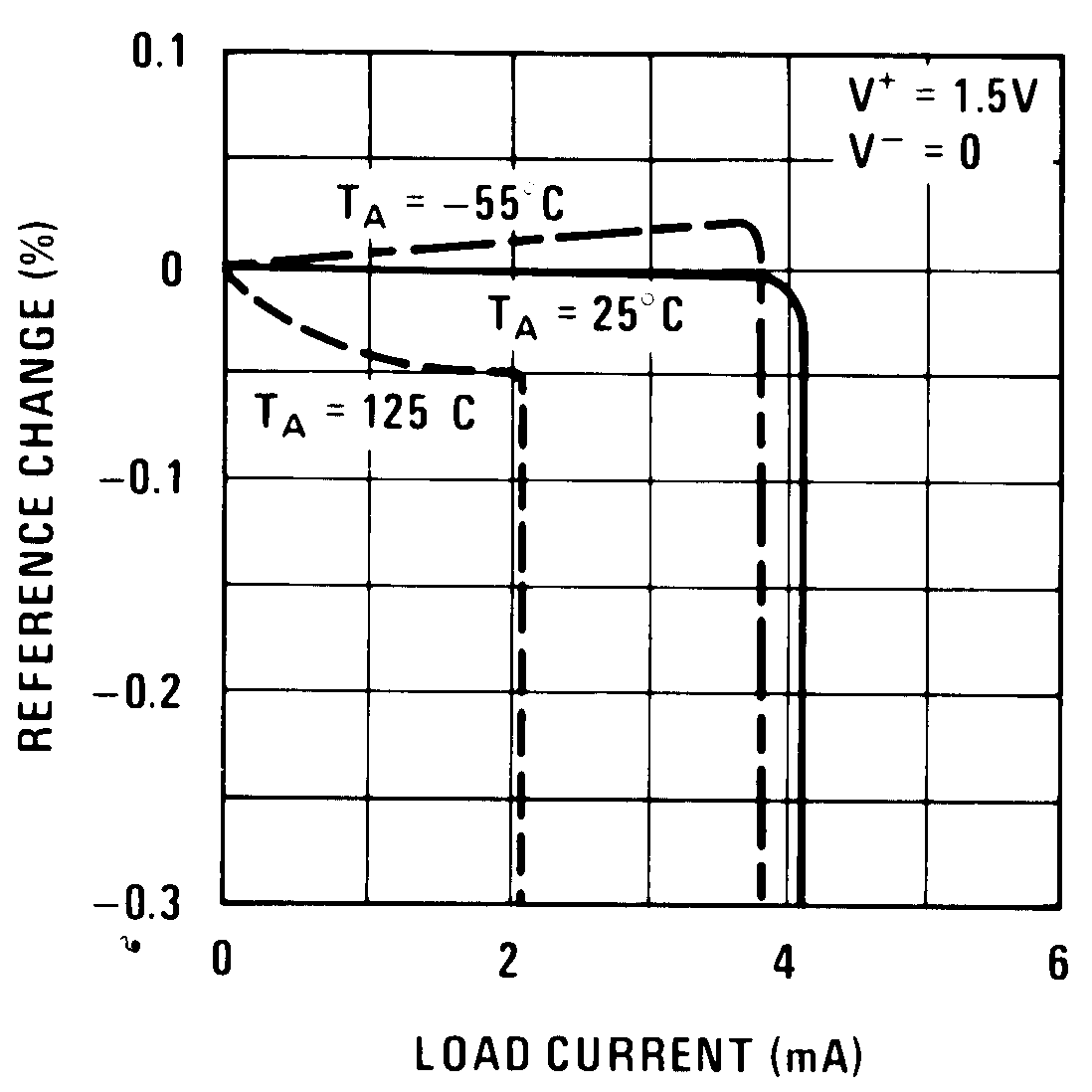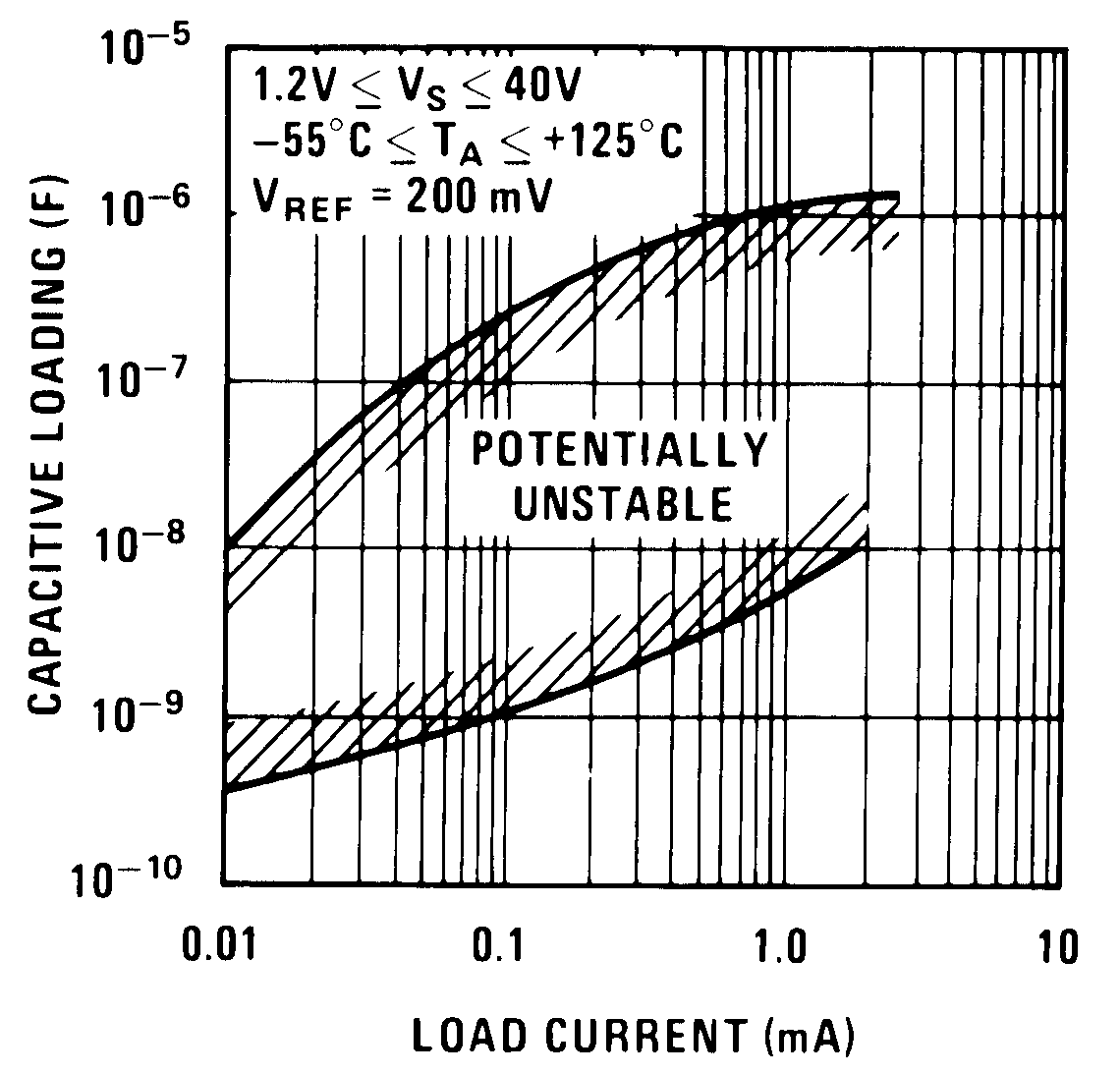SNOSBH4E May   1998  – October 2015

PRODUCTION DATA.

1. Features
2. Applications
3. Description
4. Revision History
5. Pin Configuration and Functions
6. Specifications
7. Detailed Description
1. 7.1 Overview
2. 7.2 Functional Block Diagram
3. 7.3 Feature Description
4. 7.4 Device Functional Modes
8. Application and Implementation
1. 8.1 Application Information
2. 8.2 Typical Application
3. 8.3 System Examples
9. Power Supply Recommendations
10. 10Layout
11. 11Device and Documentation Support
1. 11.1 Device Support
1. 11.1.1 Device Nomenclature
2. 11.2 Documentation Support
3. 11.3 Community Resources
5. 11.5 Electrostatic Discharge Caution
6. 11.6 Glossary
12. 12Mechanical, Packaging, and Orderable Information

• NPA|14
• P|8

## 6 Specifications

### 6.1 Absolute Maximum Ratings

See (5)(1)(6)
MIN MAX UNIT
Total supply voltage LM10/LM10B/LM10C 45 V
LM10BL/LM10CL 7 V
Differential input voltage(2) LM10/LM10B/LM10C ±40 V
LM10BL/LM10CL ±7 V
Power dissipation(3) Internally limited
Output short-circuit duration(4) Continuous
Lead temperature TO Soldering (10 seconds) 300 °C
DIP Soldering (10 seconds)  260 °C
Vapor phase (60 seconds) 215 °C
Infrared (15 seconds) 220 °C
Maximum junction temperature LM10 150 °C
LM10B 100 °C
LM10C 85 °C
Storage temperature, Tstg −55 150 °C
(1) Stresses beyond those listed under Absolute Maximum Ratings may cause permanent damage to the device. These are stress ratings only, and functional operation of the device at these or any other conditions beyond those indicated under Recommended Operating Conditions is not implied. Exposure to absolute-maximum-rated conditions for extended periods may affect device reliability.
(2) The Input voltage can exceed the supply voltages provided that the voltage from the input to any other terminal does not exceed the maximum differential input voltage and excess dissipation is accounted for when VIN < V.
(3) The maximum, operating-junction temperature is 150°C for the LM10, 100°C for the LM10B(L) and 85°C for the LM10C(L). At elevated temperatures, devices must be derated based on package thermal resistance.
(4) Internal thermal limiting prevents excessive heating that could result in sudden failure, but the IC can be subjected to accelerated stress with a shorted output and worst-case conditions.
(5) Refer to RETS10X for LM10H military specifications.
(6) If Military/Aerospace specified devices are required, please contact the TI Sales Office/Distributors for availability and specifications.

### 6.2 Recommended Operating Conditions

over operating free-air temperature range (unless otherwise noted)
MIN NOM MAX UNIT
VS Supply input voltage range (V–) – (V+) 1.2 40 V
VCM Common-mode voltage (V–) (V+) – 0.85 V
VREF Reference voltage 0.2 V
IREF Reference current 0 1 mA

### 6.3 Thermal Information

THERMAL METRIC(1) LM10 UNIT
NEV (SDIP) NPA (SOIC) P (PDIP)
8 PINS 14 PINS 8 PINS
RθJA Junction-to-ambient thermal resistance 150 90 87 °C/W
RθJC(top) Junction-to-case (top) thermal resistance 45 °C/W

### 6.4 Electrical Characteristics LM10/LM10B

TJ=25°C unless otherwise specified(1)
PARAMETER TEST CONDITIONS MIN TYP MAX UNIT
Input offset voltage TJ=25°C 0.3 2 mV
TMIN ≤ TJ ≤ TMAX (see (1)) 3 mV
Input offset current(2) TJ=25°C 0.25 0.7 nA
TMIN ≤ TJ ≤ TMAX (see (1)) 1.5 nA
Input bias current TJ=25°C 10 20 nA
TMIN ≤ TJ ≤ TMAX (see (1)) 30 nA
Input resistance TJ=25°C 250 500
TMIN ≤ TJ ≤ TMAX (see (1)) 150
Large signal voltage gain VS = ±20 V, IOUT = 0 120 400 V/mV
VOUT = ±19.95 V, TMIN ≤ TJ ≤ TMAX (see (1)) 80 V/mV
VS = ±20 V, VOUT = ±19.4 V 50 130 V/mV
IOUT = ±20 mA, TMIN ≤ TJ ≤ TMAX (see (1)) 20 V/mV
IOUT = ±15 mA, TMIN ≤ TJ ≤ TMAX (see (1)) 20 V/mV
VS = ±0.6 V, IOUT = ±2 mA 1.5 3 V/mV
VS = ±0.65 V, IOUT = ±2 mA, TMIN ≤ TJ ≤ TMAX (see (1)) 1.5 3 V/mV
VOUT = ±0.4 V, TMIN ≤ TJ ≤ TMAX (see (1)) 0.5 V/mV
VOUT = ±0.3 V, VCM = −0.4 V, TMIN ≤ TJ ≤ TMAX (see (1)) 0.5 V/mV
Shunt gain(3) 1.2 V ≤ VOUT ≤ 40 V, RL = 1.1 kΩ 14 33 V/mV
1.3 V ≤ VOUT ≤ 40 V, RL = 1.1 kΩ, TMIN ≤ TJ ≤ TMAX (see (1)) 14 33 V/mV
0.1 mA ≤ IOUT ≤ 5 mA, TMIN ≤ TJ ≤ TMAX (see (1)) 6 V/mV
1.5 V ≤ V+ ≤ 40 V, RL = 250 Ω 8 25 V/mV
0.1 mA ≤ IOUT ≤ 20 mA, TMIN ≤ TJ ≤ TMAX (see (1)) 4 V/mV
Common-mode rejection −20 V ≤ VCM ≤ 19.15 V 93 102 dB
−20 V ≤ VCM ≤ 19 V, TMIN ≤ TJ ≤ TMAX (see (1)) 93 102 dB
VS = ±20 V, TMIN ≤ TJ ≤ TMAX (see (1)) 87 dB
Supply-voltage rejection −0.2 V ≥ V ≥ −39 V 90 96 dB
V+ = 1 V, TMIN ≤ TJ ≤ TMAX (see (1)) 84 dB
V+ = 1.1 V, TMIN ≤ TJ ≤ TMAX (see (1)) 84 dB
1 V ≤ V+ ≤ 39.8 V 96 106 dB
1.1 V ≤ V+ ≤ 39.8 V, TMIN ≤ TJ ≤ TMAX (see (1)) 96 106 dB
V = −0.2 V, TMIN ≤ TJ ≤ TMAX (see (1)) 90 dB
Offset voltage drift 2 μV/°C
Offset current drift 2 pA/°C
Bias current drift TC < 100°C 60 pA/°C
Line regulation 1.2 V ≤ VS ≤ 40 V 0.001 0.003 %/V
1.3 V ≤ VS ≤ 40 V, TMIN ≤ TJ ≤ TMAX (see (1)) 0.001 0.003 %/V
0 ≤ IREF ≤ 1 mA, VREF = 200 mV, TMIN ≤ TJ ≤ TMAX (see (1)) 0.006 %/V
Load regulation 0 ≤ IREF ≤ 1 mA 0.01% 0.1%
V+− VREF ≥ 1 V, TMIN ≤ TJ ≤ TMAX (see (1)) 0.15%
V+− VREF ≥ 1.1 V, TMIN ≤ TJ ≤ TMAX (see (1)) 0.15%
Amplifier gain 0.2 V ≤ VREF ≤ 35 V TJ=25°C 50 75 V/mV
TMIN ≤ TJ ≤ TMAX (see (1)) 23 V/mV
Feedback sense voltage TJ=25°C 195 200 205 mV
TMIN ≤ TJ ≤ TMAX (see (1)) 194 206 mV
Feedback current TJ=25°C 20 50 nA
TMIN ≤ TJ ≤ TMAX (see (1)) 65 nA
Reference drift 0.002 %/°C
Supply current TJ=25°C 270 400 μA
TMIN ≤ TJ ≤ TMAX (see (1)) 500 μA
Supply current change 1.2 V ≤ VS ≤ 40 V TJ=25°C 15 μA
TMIN ≤ TJ ≤ TMAX (see (1)) 75
1.3 V ≤ VS ≤ 40 V TJ=25°C 15 μA
TMIN ≤ TJ ≤ TMAX (see (1)) 75
(1) These specifications apply for V ≤ VCM ≤ V+− 0.85 V, 1 V (TMIN ≤ TJ ≤ TMAX), 1.2 V, 1.3 V (TMIN ≤ TJ ≤ TMAX) < VS ≤ VMAX, VREF = 0.2 V and 0 ≤ IREF ≤ 1 mA, unless otherwise specified: VMAX = 40 V for the standard part and 6.5 V for the low voltage part. The full-temperature-range operation is −55°C to 125°C for the LM10, −25°C to 85°C for the LM10B(L) and 0°C to 70°C for the LM10C(L). The specifications do not include the effects of thermal gradients (τ1 ≃ 20 ms), die heating (τ2 ≃ 0.2 s) or package heating. Gradient effects are small and tend to offset the electrical error (see curves).
(2) For TJ > 90°C, IOS may exceed 1.5 nA for VCM = V. With TJ = 125°C and V ≤ VCM ≤ V + 0.1 V, IOS ≤ 5 nA.
(3) This defines operation in floating applications such as the bootstrapped regulator or two-wire transmitter. Output is connected to the V+ terminal of the IC and input common mode is referred to V (see System Examples). Effect of larger output-voltage swings with higher load resistance can be accounted for by adding the positive-supply rejection error.

### 6.5 Electrical Characteristics, LM10C

TJ=25°C unless otherwise specified(1)
PARAMETER TEST CONDITIONS MIN TYP MAX UNIT
Input offset voltage TJ=25°C 0.5 4 mV
TMIN ≤ TJ ≤ TMAX (see (1)) 5 mV
Input offset current(2) TJ=25°C 0.4 2 nA
TMIN ≤ TJ ≤ TMAX (see (1)) 3 nA
Input bias current TJ=25°C 12 30 nA
TMIN ≤ TJ ≤ TMAX (see (1)) 40 nA
Input resistance TJ=25°C 150 400
TMIN ≤ TJ ≤ TMAX (see (1)) 115
Large signal voltage gain VS = ±20 V, IOUT = 0 80 400 V/mV
VOUT = ±19.95 V, TMIN ≤ TJ ≤ TMAX (see (1)) 50 V/mV
VS = ±20 V, VOUT = ±19.4 V 25 130 V/mV
IOUT = ±20 mA, TMIN ≤ TJ ≤ TMAX (see (1)) 15 V/mV
IOUT = ±15 mA, TMIN ≤ TJ ≤ TMAX (see (1)) 15 V/mV
VS = ±0.6 V, IOUT = ±2 mA 1 3 V/mV
VS = 0.65 V, IOUT = ±2 mA, TMIN ≤ TJ ≤ TMAX (see (1)) 1 3 V/mV
VOUT = ±0.4 V, TMIN ≤ TJ ≤ TMAX (see (1)) 0.75 V/mV
VOUT = ±0.3 V, VCM = −0.4 V, TMIN ≤ TJ ≤ TMAX (see (1)) 0.75 V/mV
Shunt gain(3) 1.2 V ≤ VOUT ≤ 40 V, RL = 1.1 kΩ 10 33 V/mV
1.3 V ≤ VOUT ≤ 40 V, RL = 1.1 kΩ, TMIN ≤ TJ ≤ TMAX (see (1)) 10 33 V/mV
0.1 mA ≤ IOUT ≤ 5 mA, TMIN ≤ TJ ≤ TMAX (see (1)) 6 V/mV
1.5 V ≤ V+ ≤ 40 V, RL = 250 Ω 6 25 V/mV
0.1 mA ≤ IOUT ≤ 20 mA, TMIN ≤ TJ ≤ TMAX (see (1)) 4 V/mV
Common-mode rejection −20 V ≤ VCM ≤ 19.15 V 90 102 dB
−20 V ≤ VCM ≤ 19 V 90 102 dB
VS = ±20 V, TMIN ≤ TJ ≤ TMAX (see (1)) 87 dB
Supply-voltage rejection −0.2 V ≥ V ≥ −39 V 87 96 dB
V+ = 1 V, TMIN ≤ TJ ≤ TMAX (see (1)) 84 dB
V+ = 1.1 V, TMIN ≤ TJ ≤ TMAX (see (1)) 84 dB
1 V ≤ V+ ≤ 39.8 V 93 106 dB
1.1 V ≤ V+ ≤ 39.8 V, TMIN ≤ TJ ≤ TMAX (see (1)) 93 106 dB
V = −0.2 V, TMIN ≤ TJ ≤ TMAX (see (1)) 90 dB
Offset voltage drift 5 μV/°C
Offset current drift 5 pA/°C
Bias current drift TC < 100°C 90 pA/°C
Line regulation 1.2 V ≤ VS ≤ 40 V 0.001 0.008 %/V
1.3 V ≤ VS ≤ 40 V, TMIN ≤ TJ ≤ TMAX (see (1)) 0.001 0.008 %/V
0 ≤ IREF ≤ 1 mA, VREF = 200 mV, TMIN ≤ TJ ≤ TMAX (see (1)) 0.01 %/V
Load regulation 0 ≤ IREF ≤ 1 mA 0.01% 0.15%
V+ − VREF ≥ 1 V, TMIN ≤ TJ ≤ TMAX (see (1)) 0.2%
V+ − VREF≥ 1.1 V, TMIN ≤ TJ ≤ TMAX (see (1)) 0.2%
Amplifier gain 0.2 V ≤ VREF ≤ 35 V TJ=25°C 25 70 V/mV
TMIN ≤ TJ ≤ TMAX (see (1)) 15 V/mV
Feedback sense voltage TJ=25°C 190 200 210 mV
TMIN ≤ TJ ≤ TMAX (see (1)) 189 211 mV
Feedback current TJ=25°C 22 75 nA
TMIN ≤ TJ ≤ TMAX (see (1)) 90 nA
Reference drift 0.003 %/°C
Supply current TJ=25°C 300 500 μA
TMIN ≤ TJ ≤ TMAX (see (1)) 570 μA
Supply current change 1.2 V ≤ VS ≤ 40 V TJ=25°C 15 μA
TMIN ≤ TJ ≤ TMAX (see (1)) 75
1.3 V ≤ VS ≤ 40 V TJ=25°C 15 μA
TMIN ≤ TJ ≤ TMAX (see (1)) 75
(1) These specifications apply for V ≤ VCM ≤ V+− 0.85 V, 1 V (TMIN ≤ TJ ≤ TMAX), 1.2 V, 1.3 V (TMIN ≤ TJ ≤ TMAX) < VS ≤ VMAX, VREF = 0.2 V and 0 ≤ IREF ≤ 1 mA, unless otherwise specified: VMAX = 40 V for the standard part and 6.5 V for the low voltage part. The full-temperature-range operation is −55°C to 125°C for the LM10, −25°C to 85°C for the LM10B(L) and 0°C to 70°C for the LM10C(L). The specifications do not include the effects of thermal gradients (τ1 ≃ 20 ms), die heating (τ2 ≃ 0.2 s) or package heating. Gradient effects are small and tend to offset the electrical error (see curves).
(2) For TJ > 90°C, IOS may exceed 1.5 nA for VCM = V. With TJ = 125°C and V ≤ VCM ≤ V + 0.1 V, IOS ≤ 5 nA.
(3) This defines operation in floating applications such as the bootstrapped regulator or two-wire transmitter. Output is connected to the V+ terminal of the IC and input common mode is referred to V (see System Examples). Effect of larger output-voltage swings with higher load resistance can be accounted for by adding the positive-supply rejection error.

### 6.6 Electrical Characteristics, LM10BL

TJ=25°C unless otherwise specified.(1)
PARAMETER TEST CONDITIONS MIN TYP MAX UNIT
Input offset voltage TJ=25°C 0.3 2 mV
TMIN ≤ TJ ≤ TMAX (see (1)) 3 mV
Input offset current(2) TJ=25°C 0.1 0.7 nA
TMIN ≤ TJ ≤ TMAX (see (1)) 1.5 nA
Input bias current TJ=25°C 10 20 nA
TMIN ≤ TJ ≤ TMAX (see (1)) 30 nA
Input resistance TJ=25°C 250 500
TMIN ≤ TJ ≤ TMAX (see (1)) 150
Large signal voltage gain VS = ±3.25 V, IOUT = 0 60 300 V/mV
VOUT = ±3.2 V, TMIN ≤ TJ ≤ TMAX (see (1)) 40 V/mV
VS = ±3.25 V, IOUT = 10 mA 10 25 V/mV
VOUT = ±2.75 V, TMIN ≤ TJ ≤ TMAX (see (1)) 4 V/mV
VS = ±0.6 V, IOUT = ±2 mA 1.5 3 V/mV
VS = 0.65 V, IOUT = ±2 mA, TMIN ≤ TJ ≤ TMAX (see (1)) 1.5 3 V/mV
VOUT = ±0.4 V, VCM = −0.4 V, TMIN ≤ TJ ≤ TMAX (see (1)) 0.5 V/mV
VOUT = ±0.3 V, VCM = −0.4 V, TMIN ≤ TJ ≤ TMAX (see (1)) 0.5 V/mV
Shunt gain(3) 1.5 V ≤ V +≤ 6.5 V, RL = 500 Ω 8 30 V/mV
0.1 mA ≤ IOUT ≤ 10 mA, TMIN ≤ TJ ≤ TMAX (see (1)) 4 V/mV
Common-mode rejection −3.25 V ≤ VCM ≤ 2.4 V 89 102 dB
−3.25 V ≤ VCM ≤ 2.25 V, TMIN ≤ TJ ≤ TMAX (see (1))
VS = ±3.25 V, TMIN ≤ TJ ≤ TMAX (see (1)) 83 dB
Supply-voltage rejection −0.2 V ≥ V ≥ −5.4 V 86 96 dB
V+ = 1 V, TMIN ≤ TJ ≤ TMAX (see (1)) 80 dB
V+ = 1.2 V, TMIN ≤ TJ ≤ TMAX (see (1)) 80 dB
1 V ≤ V+ ≤ 6.3 V 94 106 dB
1.1 V ≤ V+ ≤ 6.3 V, TMIN ≤ TJ ≤ TMAX (see (1)) 94 106 dB
V=0.2 V, TMIN ≤ TJ ≤ TMAX (see (1)) 88 dB
Offset voltage drift 2 μV/°C
Offset current drift 2 pA/°C
Bias current drift 60 pA/°C
Line regulation 1.2 V ≤ VS ≤ 6.5 V 0.001 0.01 %/V
1.3 V ≤ VS ≤ 6.5 V, TMIN ≤ TJ ≤ TMAX (see (1)) 0.001 0.01 %/V
0 ≤ IREF ≤ 0.5 mA, VREF = 200 mV, TMIN ≤ TJ ≤ TMAX (see (1)) 0.02 %/V
Load regulation 0 ≤ IREF ≤ 0.5 mA 0.01% 0.1%
V+ − VREF ≥ 1 V, TMIN ≤ TJ ≤ TMAX (see (1)) 0.15%
V+− VREF ≥ 1.1 V, TMIN ≤ TJ ≤ TMAX (see (1)) 0.15%
Amplifier gain 0.2 V ≤ VREF ≤ 5.5 V TJ=25°C 30 70 V/mV
TMIN ≤ TJ ≤ TMAX (see (1)) 20 V/mV
Feedback sense voltage TJ=25°C 195 200 205 mV
TMIN ≤ TJ ≤ TMAX (see (1)) 194 206 mV
Feedback current TJ=25°C 20 50 nA
TMIN ≤ TJ ≤ TMAX (see (1)) 65 nA
Reference drift 0.002 %/°C
Supply current TJ=25°C 260 400 μA
TMIN ≤ TJ ≤ TMAX (see (1)) 500 μA
(1) These specifications apply for V ≤ VCM ≤ V+− 0.85 V, 1 V (TMIN ≤ TJ ≤ TMAX), 1.2 V, 1.3 V (TMIN ≤ TJ ≤ TMAX) < VS ≤ VMAX, VREF = 0.2 V and 0 ≤ IREF ≤ 1 mA, unless otherwise specified: VMAX = 40 V for the standard part and 6.5 V for the low voltage part. The full-temperature-range operation is −55°C to 125°C for the LM10, −25°C to 85°C for the LM10B(L) and 0°C to 70°C for the LM10C(L). The specifications do not include the effects of thermal gradients (τ1 ≃ 20 ms), die heating (τ2 ≃ 0.2 s) or package heating. Gradient effects are small and tend to offset the electrical error (see curves).
(2) For TJ > 90°C, IOS may exceed 1.5 nA for VCM = V. With TJ=125°C and V ≤ VCM ≤ V+ 0.1 V, IOS ≤ 5 nA.
(3) This defines operation in floating applications such as the bootstrapped regulator or two-wire transmitter. Output is connected to the V+ terminal of the IC and input common mode is referred to V (see System Examples). Effect of larger output-voltage swings with higher load resistance can be accounted for by adding the positive-supply rejection error.

### 6.7 Electrical Characteristics, LM10CL

TJ=25°C unless otherwise specified.(1)
PARAMETER TEST CONDITIONS MIN TYP MAX UNIT
Input offset voltage TJ=25°C 0.5 4 mV
TMIN ≤ TJ ≤ TMAX (see (1)) 5 mV
Input offset current(2) TJ=25°C 0.2 2 nA
TMIN ≤ TJ ≤ TMAX (see (1)) 3 nA
Input bias current TJ=25°C 12 30 nA
TMIN ≤ TJ ≤ TMAX (see (1)) 40 nA
Input resistance TJ=25°C 150 400
TMIN ≤ TJ ≤ TMAX (see (1)) 115
Large signal voltage gain VS = ±3.25 V, IOUT = 0 40 300 V/mV
VOUT = ±3.2 V, TMIN ≤ TJ ≤ TMAX (see (1)) 25 V/mV
VS = ±3.25 V, IOUT = 10 mA 5 25 V/mV
VOUT = ±2.75 V, TMIN ≤ TJ ≤ TMAX (see (1)) 3 V/mV
VS = ±0.6 V, IOUT = ±2 mA 1 3 V/mV
VS = 0.65 V, IOUT = ±2 mA, TMIN ≤ TJ ≤ TMAX (see (1)) 1 3 V/mV
VOUT = ±0.4 V, VCM = −0.4 V, TMIN ≤ TJ ≤ TMAX (see (1)) 0.75 V/mV
VOUT = ±0.3 V, VCM = −0.4 V, TMIN ≤ TJ ≤ TMAX (see (1)) 0.75 V/mV
Shunt gain(3) 1.5 V ≤ V+ ≤ 6.5 V, RL= 500 Ω 6 30 V/mV
0.1 mA ≤ IOUT ≤ 10 mA, TMIN ≤ TJ ≤ TMAX (see (1)) 4 V/mV
Common-mode rejection −3.25 V ≤ VCM ≤ 2.4 V 80 102 dB
−3.25 V ≤ VCM ≤ 2.25 V, TMIN ≤ TJ ≤ TMAX (see (1)) 80 102 dB
VS = ±3.25 V, TMIN ≤ TJ ≤ TMAX (see (1)) 74 dB
Supply-voltage rejection −0.2 V ≥ V ≥ −5.4 V 80 96 dB
V+ = 1 V, TMIN ≤ TJ ≤ TMAX (see (1)) 74 dB
V+ = 1.2 V, TMIN ≤ TJ ≤ TMAX (see (1)) 74 dB
1 V ≤ V+ ≤ 6.3 V 80 106 dB
1.1 V ≤ V+ ≤ 6.3 V, TMIN ≤ TJ ≤ TMAX (see (1)) 80 106 dB
V = 0.2 V, TMIN ≤ TJ ≤ TMAX (see (1)) 74 dB
Offset voltage drift 5 μV/°C
Offset current drift 5 pA/°C
Bias current drift 90 pA/°C
Line regulation 1.2 V ≤ VS ≤ 6.5 V 0.001 0.02 %/V
1.3 V ≤ VS ≤ 6.5 V, TMIN ≤ TJ ≤ TMAX (see (1)) 0.001 0.02 %/V
0 ≤ IREF ≤ 0.5 mA, VREF = 200 mV, TMIN ≤ TJ ≤ TMAX (see (1)) 0.03 %/V
Load regulation 0 ≤ IREF ≤ 0.5 mA 0.01% 0.15%
V+− VREF ≥ 1 V, TMIN ≤ TJ ≤ TMAX (see (1)) 0.2%
V+− VREF ≥ 1.1 V, TMIN ≤ TJ ≤ TMAX (see (1)) 0.2%
Amplifier gain 0.2 V ≤ VREF ≤ 5.5 V TJ=25°C 20 70 V/mV
TMIN ≤ TJ ≤ TMAX (see (1)) 15 V/mV
Feedback sense voltage TJ=25°C 190 200 210 mV
TMIN ≤ TJ ≤ TMAX (see (1)) 189 211 mV
Feedback current TJ=25°C 22 75 nA
TMIN ≤ TJ ≤ TMAX (see (1)) 90 nA
Reference drift 0.003 %/°C
Supply current TJ=25°C 280 500 μA
TMIN ≤ TJ ≤ TMAX (see (1)) 570 μA
(1) These specifications apply for V ≤ VCM ≤ V+− 0.85 V, 1 V (TMIN ≤ TJ ≤ TMAX), 1.2 V, 1.3 V (TMIN ≤ TJ ≤ TMAX) < VS ≤ VMAX, VREF = 0.2 V and 0 ≤ IREF ≤ 1 mA, unless otherwise specified: VMAX = 40 V for the standard part and 6.5 V for the low voltage part. The full-temperature-range operation is −55°C to 125°C for the LM10, −25°C to 85°C for the LM10B(L) and 0°C to 70°C for the LM10C(L). The specifications do not include the effects of thermal gradients (τ1 ≃ 20 ms), die heating (τ2 ≃ 0.2 s) or package heating. Gradient effects are small and tend to offset the electrical error (see curves).
(2) For TJ > 90°C, IOS may exceed 1.5 nA for VCM = V. With TJ = 125°C and V ≤ VCM ≤ V + 0.1 V, IOS ≤ 5 nA.
(3) This defines operation in floating applications such as the bootstrapped regulator or two-wire transmitter. Output is connected to the V+ terminal of the IC and input common mode is referred to V (see System Examples). Effect of larger output-voltage swings with higher load resistance can be accounted for by adding the positive-supply rejection error.

### 6.8 Typical Characteristics

#### 6.8.1 Typical Characteristics (Op Amp)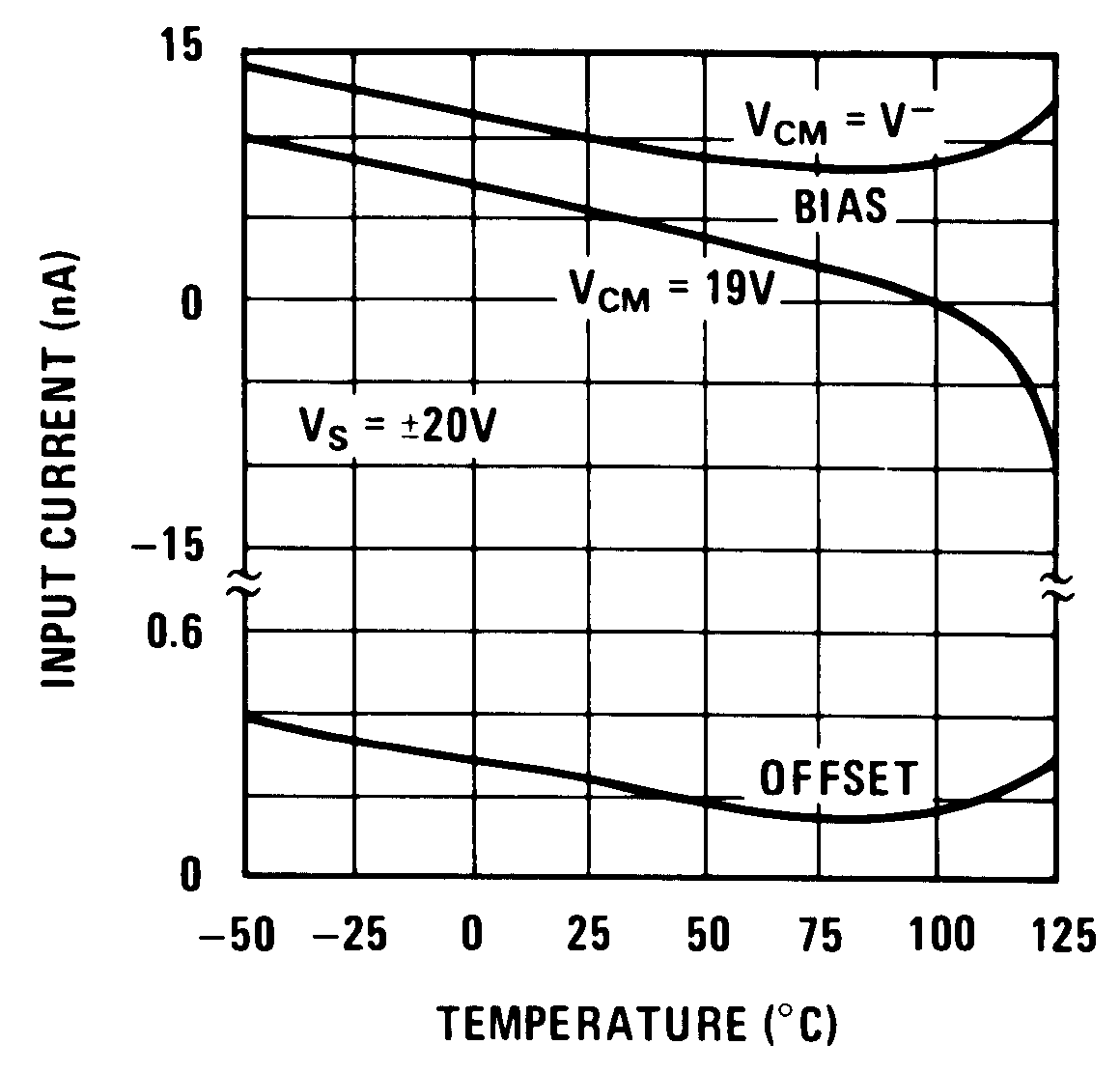Figure 1. Input Current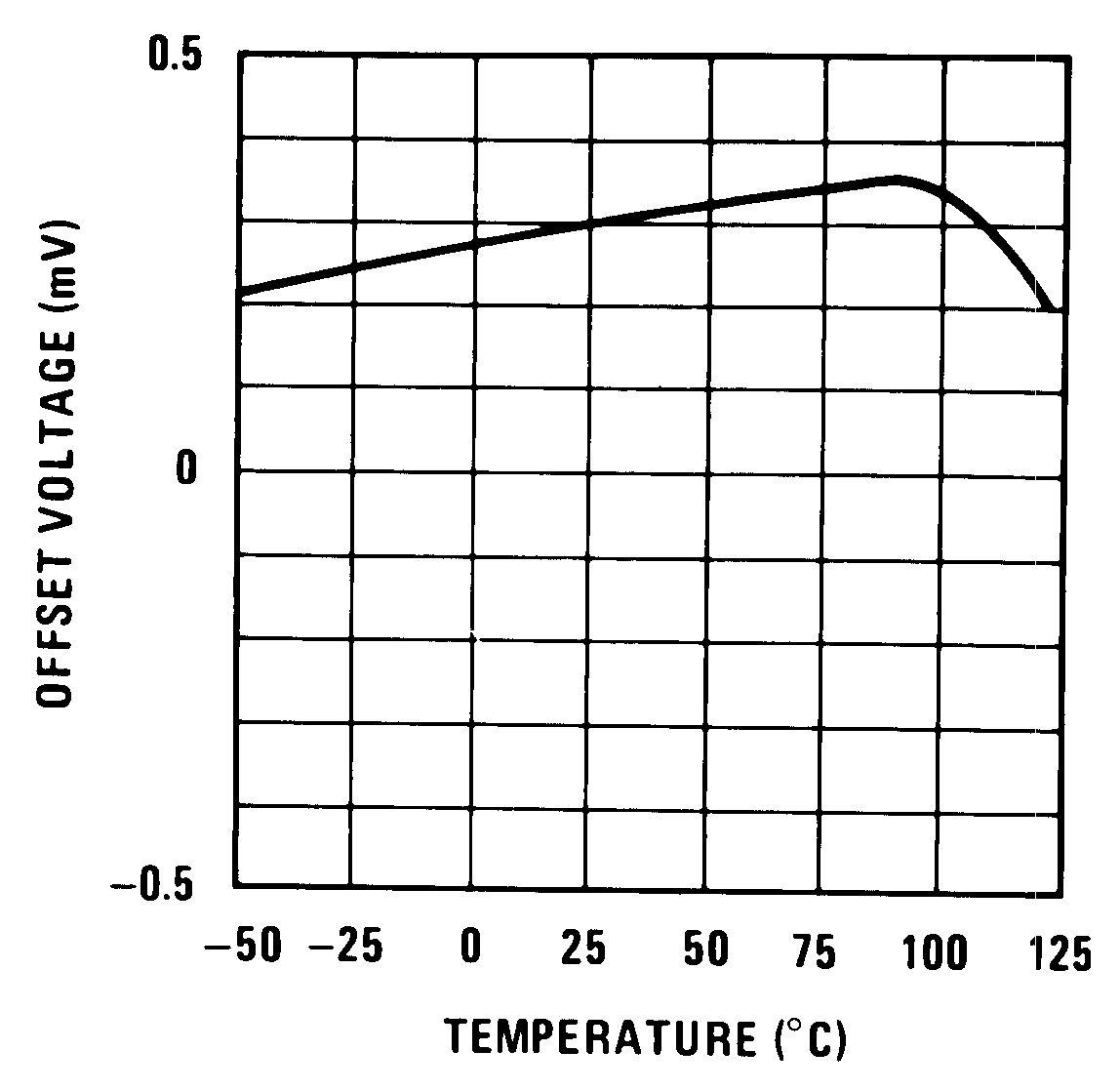Figure 3. Output Voltage Drift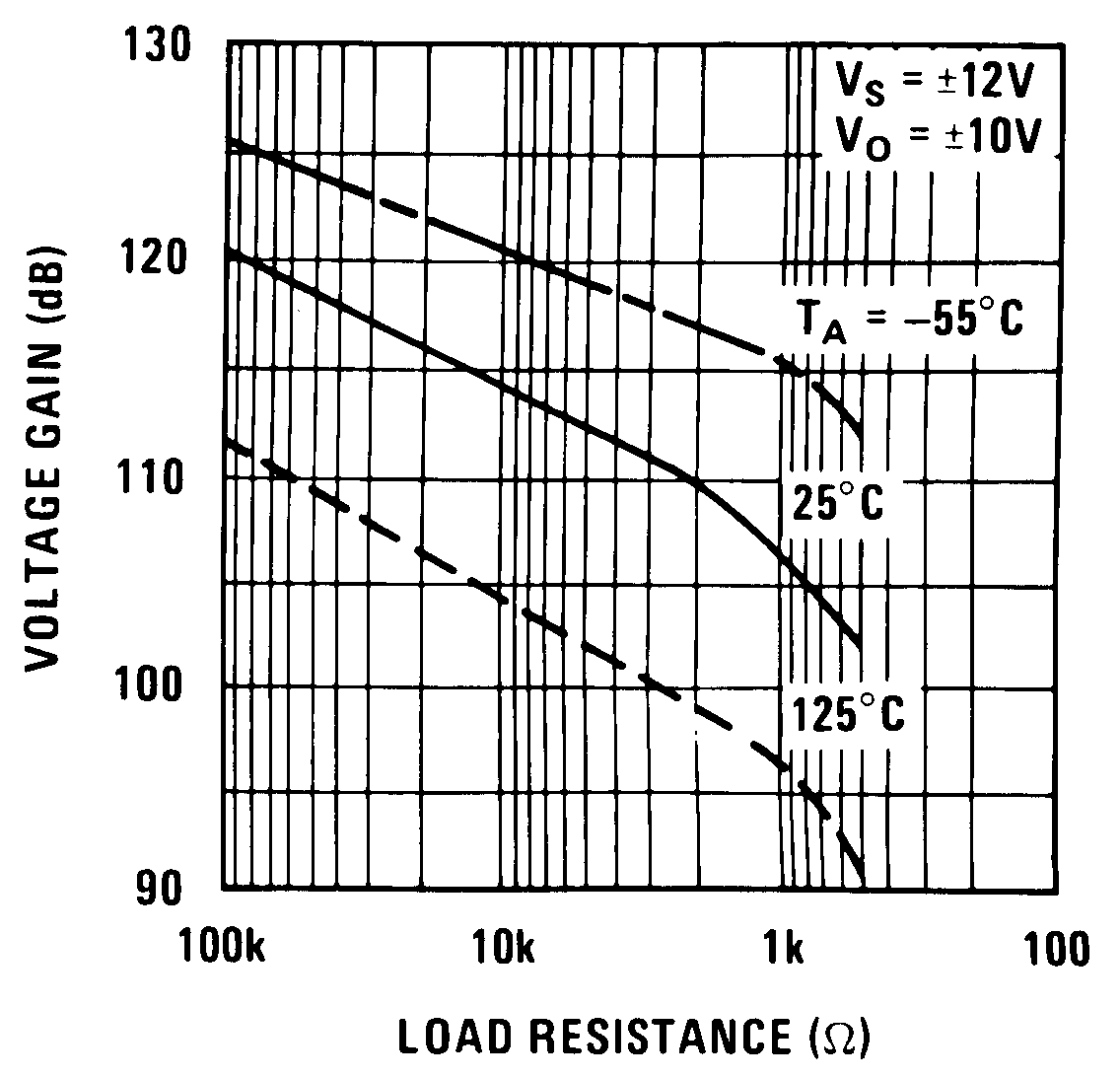Figure 5. DC Voltage Gain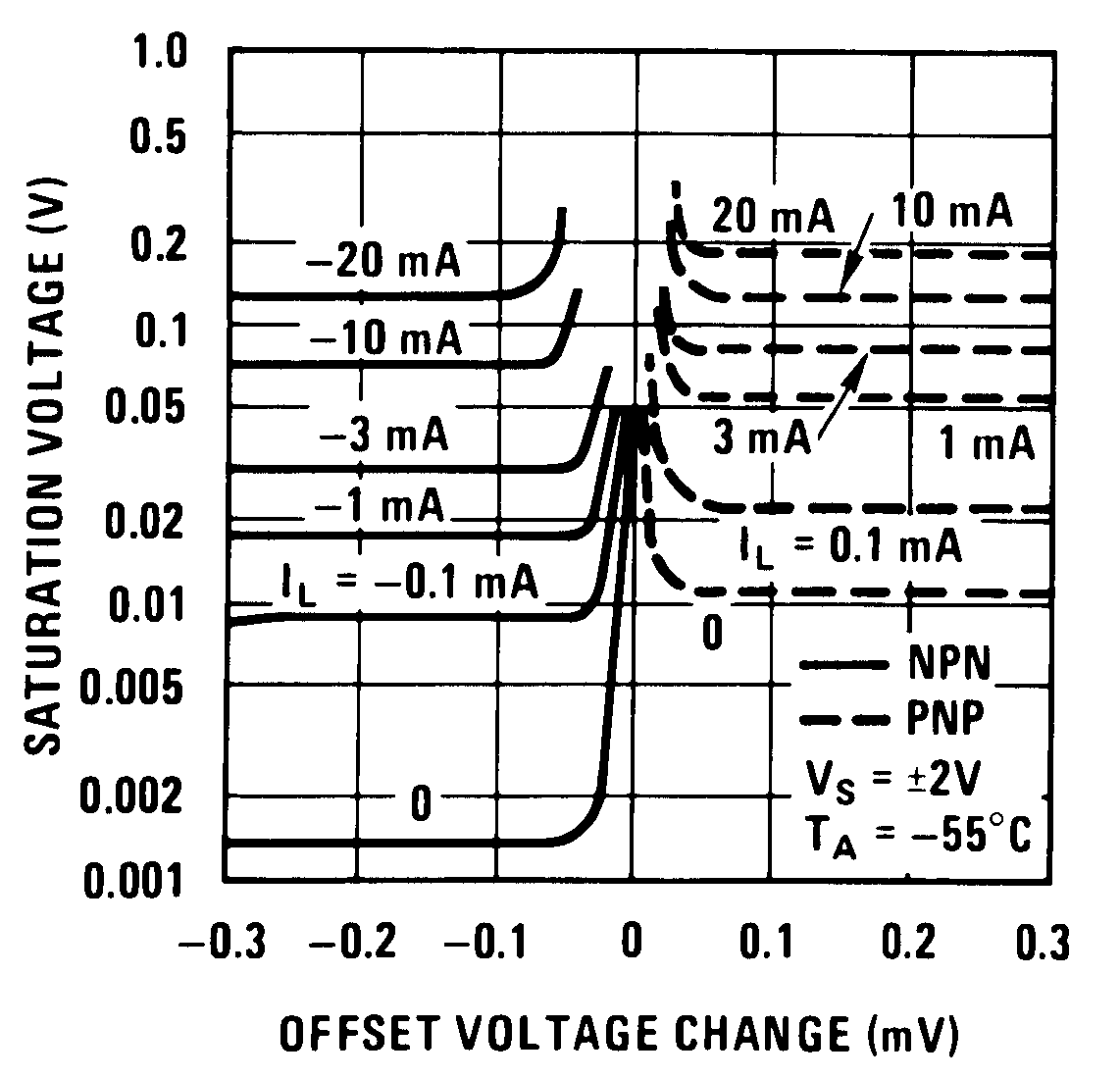Figure 7. Output Saturation Characteristics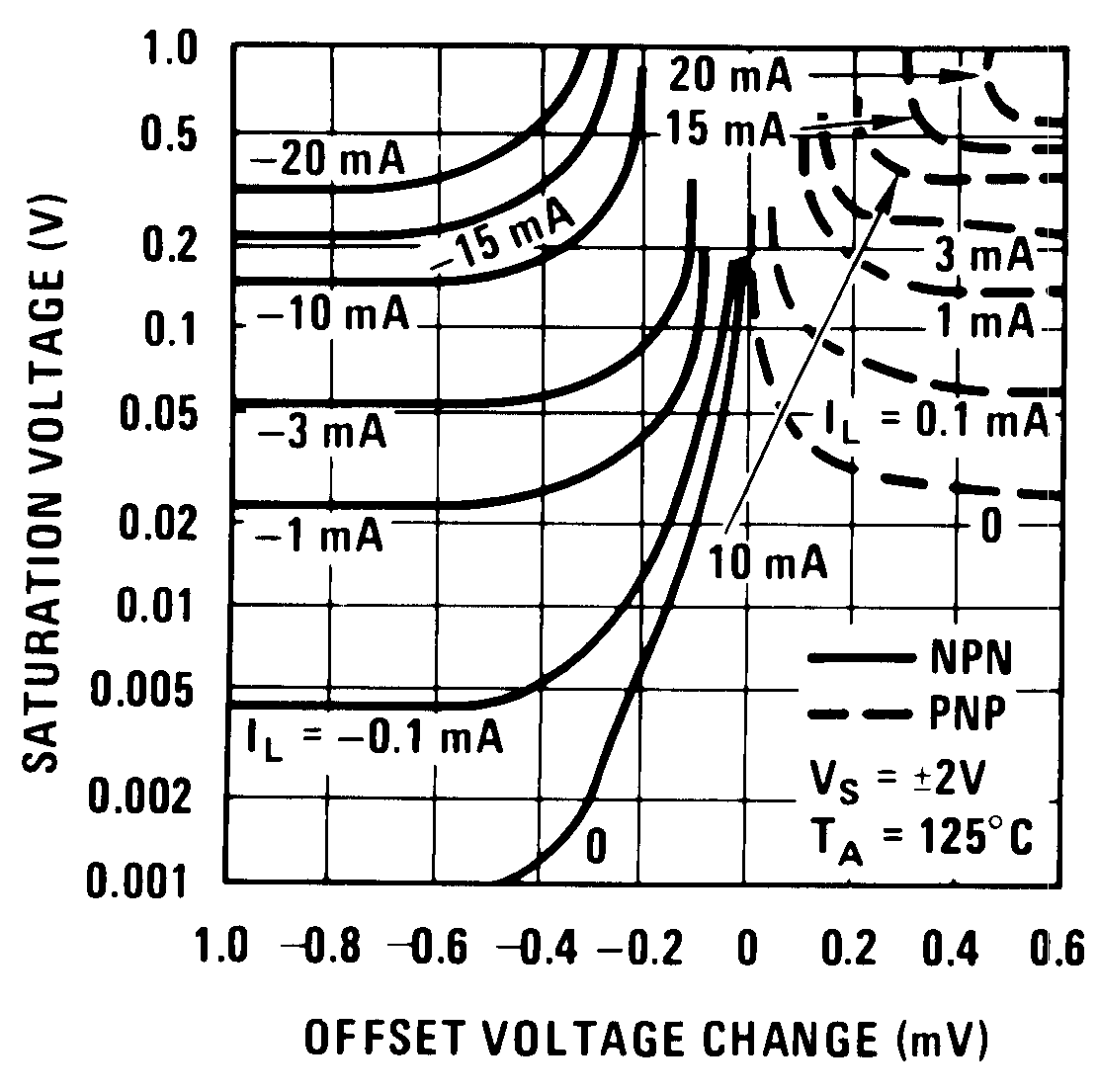Figure 9. Output Saturation Characteristics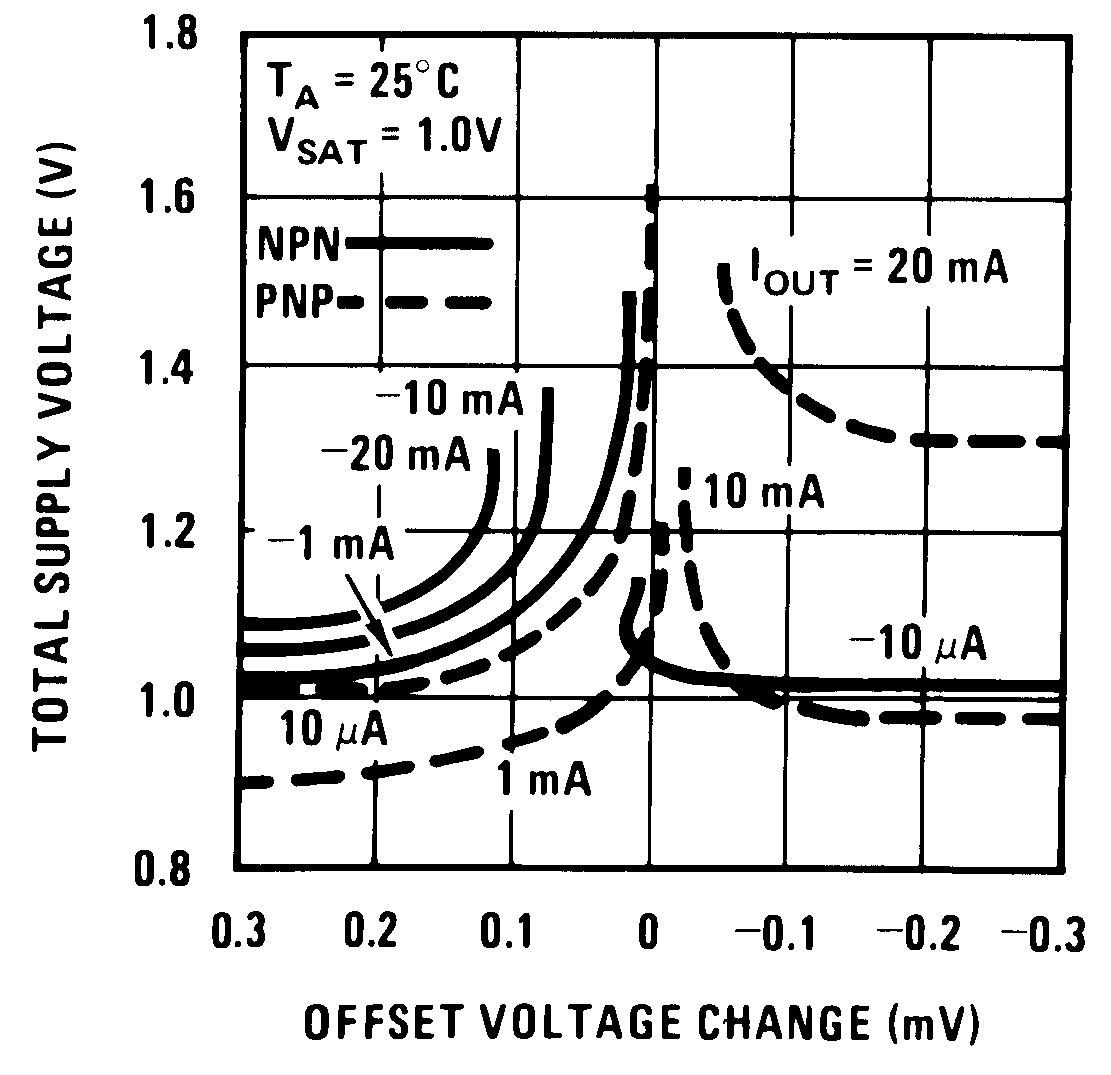Figure 11. Minimum Supply Voltage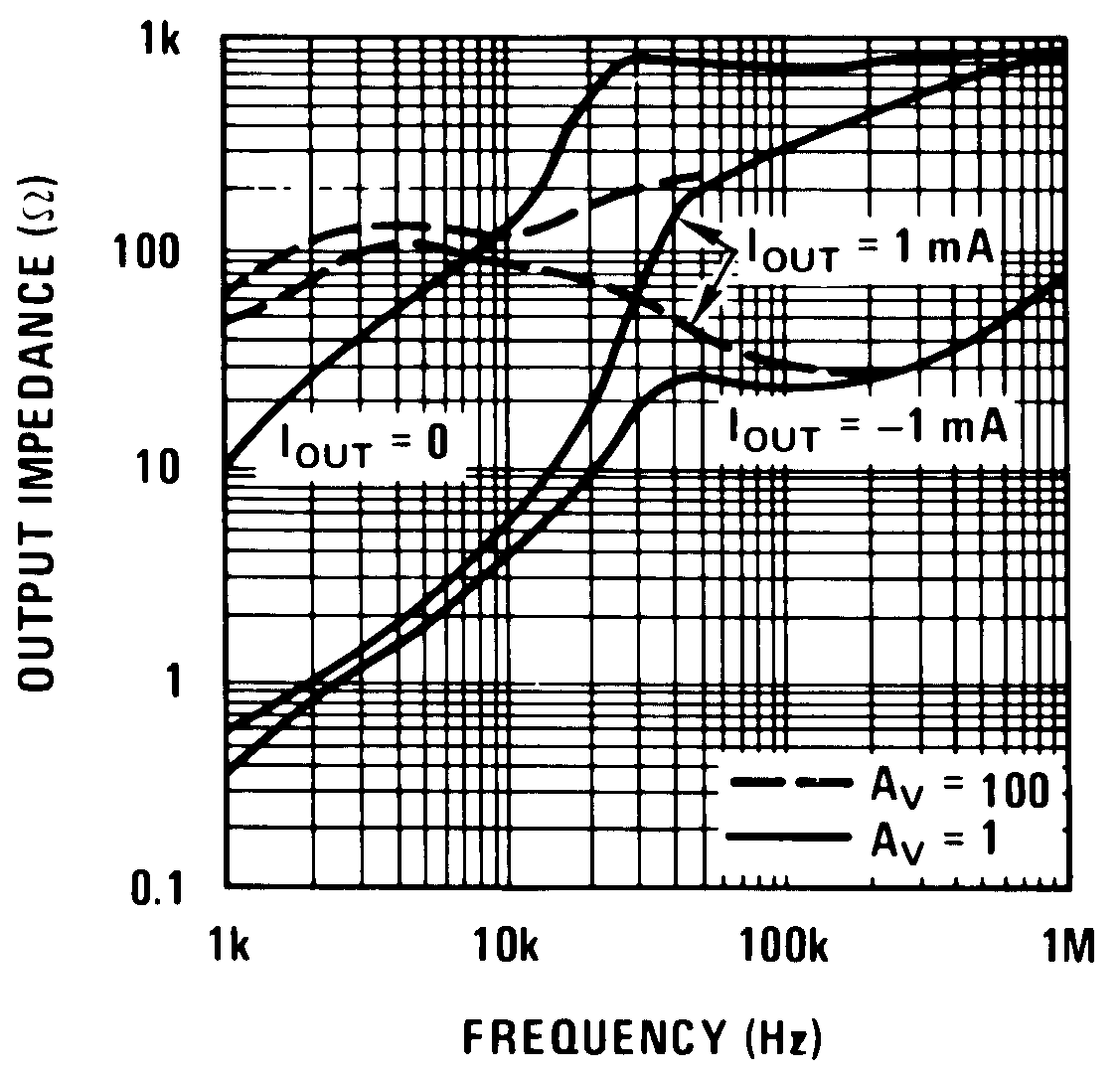Figure 13. Output ImpedanceFigure 15. Large Signal ResponseFigure 17. Comparator Response Time For Various Input Overdrives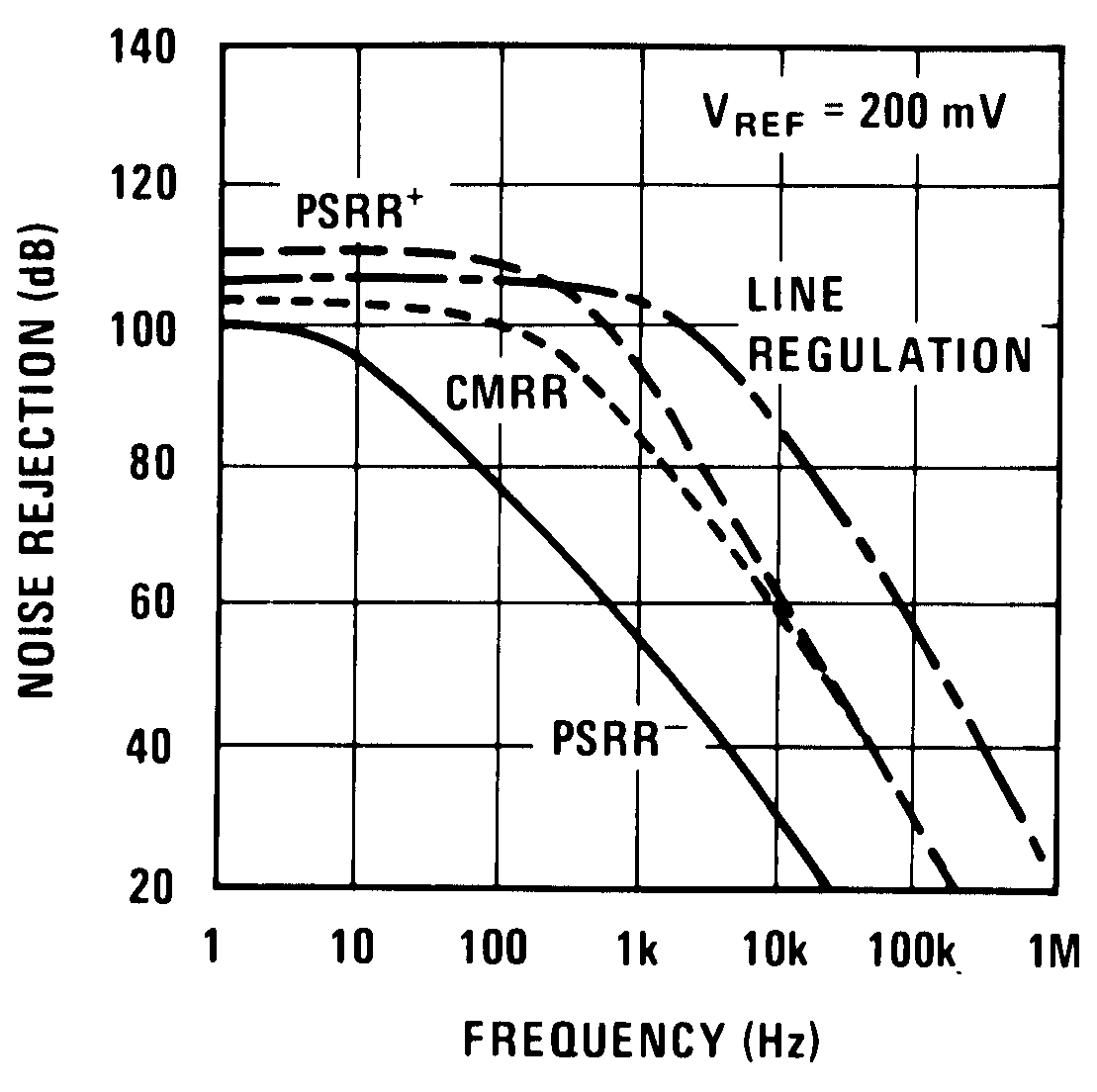Figure 19. Noise Rejection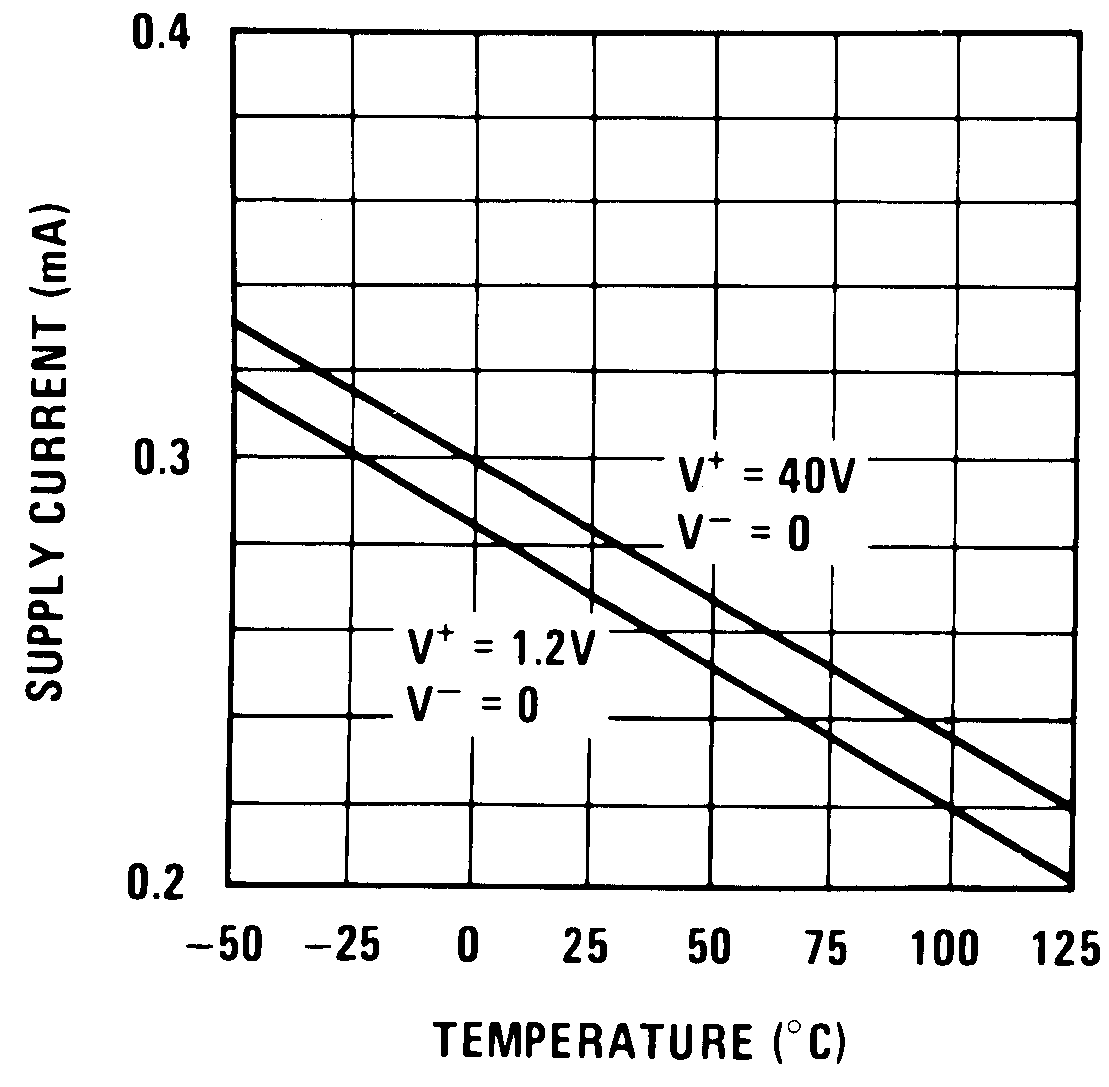Figure 21. Supply Current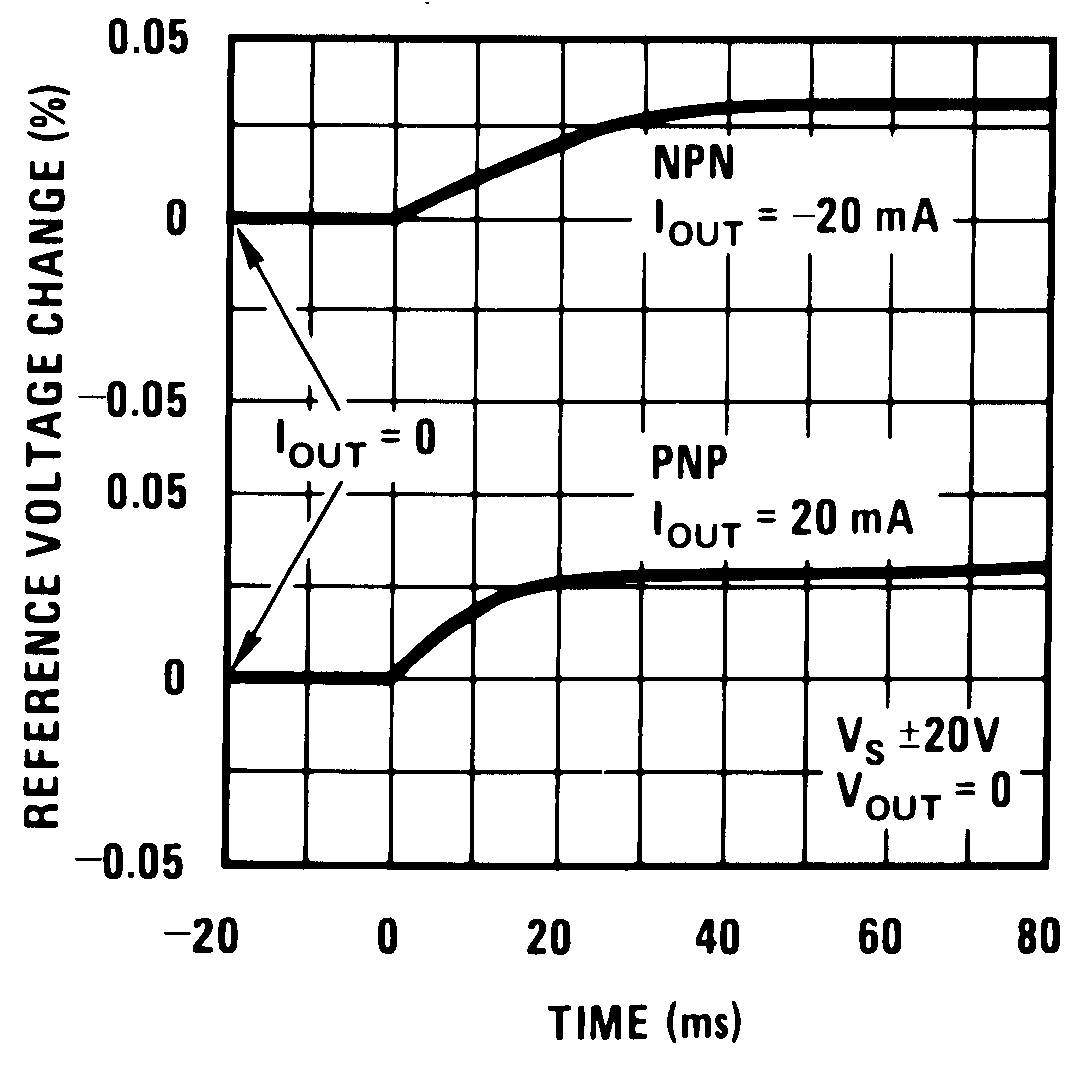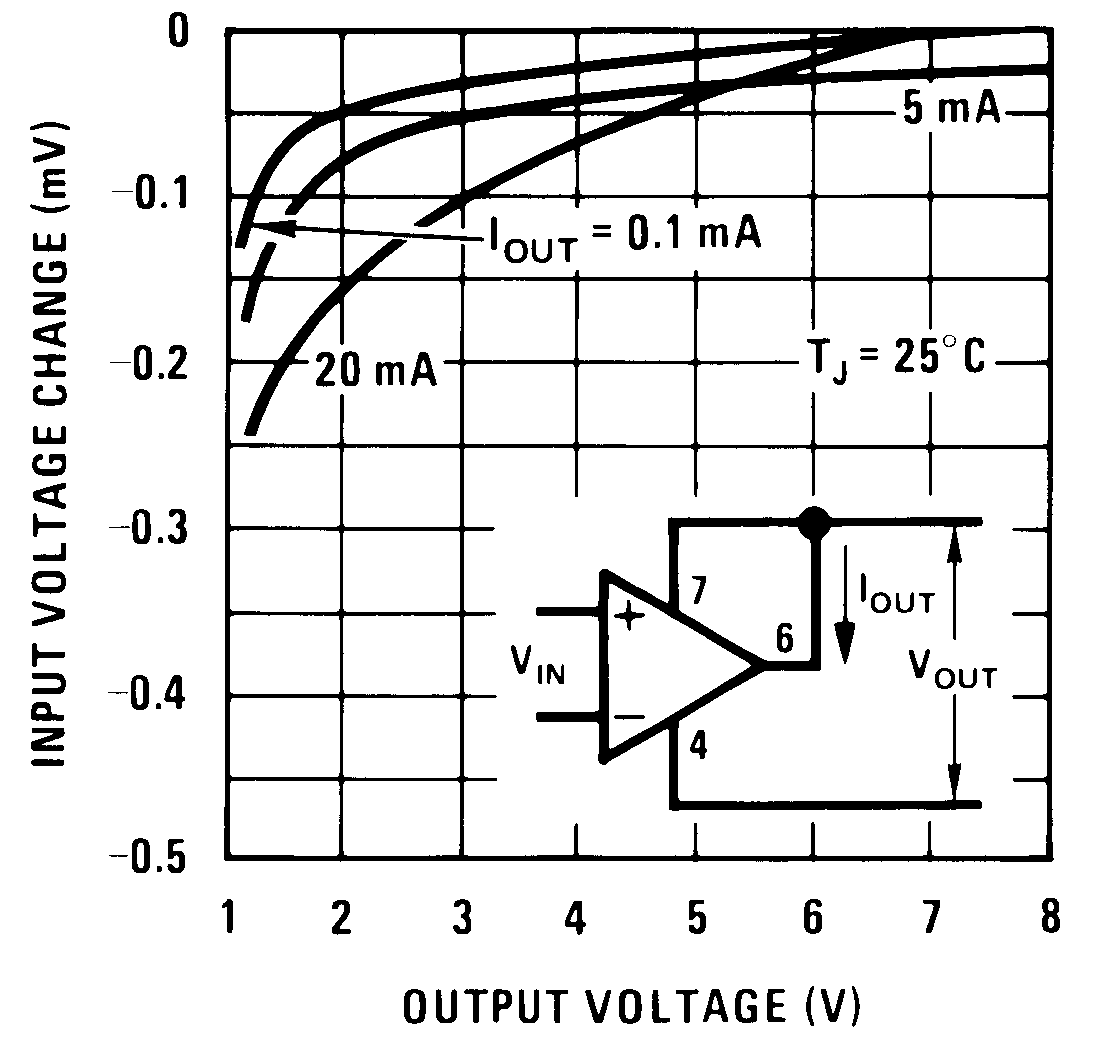Figure 25. Shunt Gain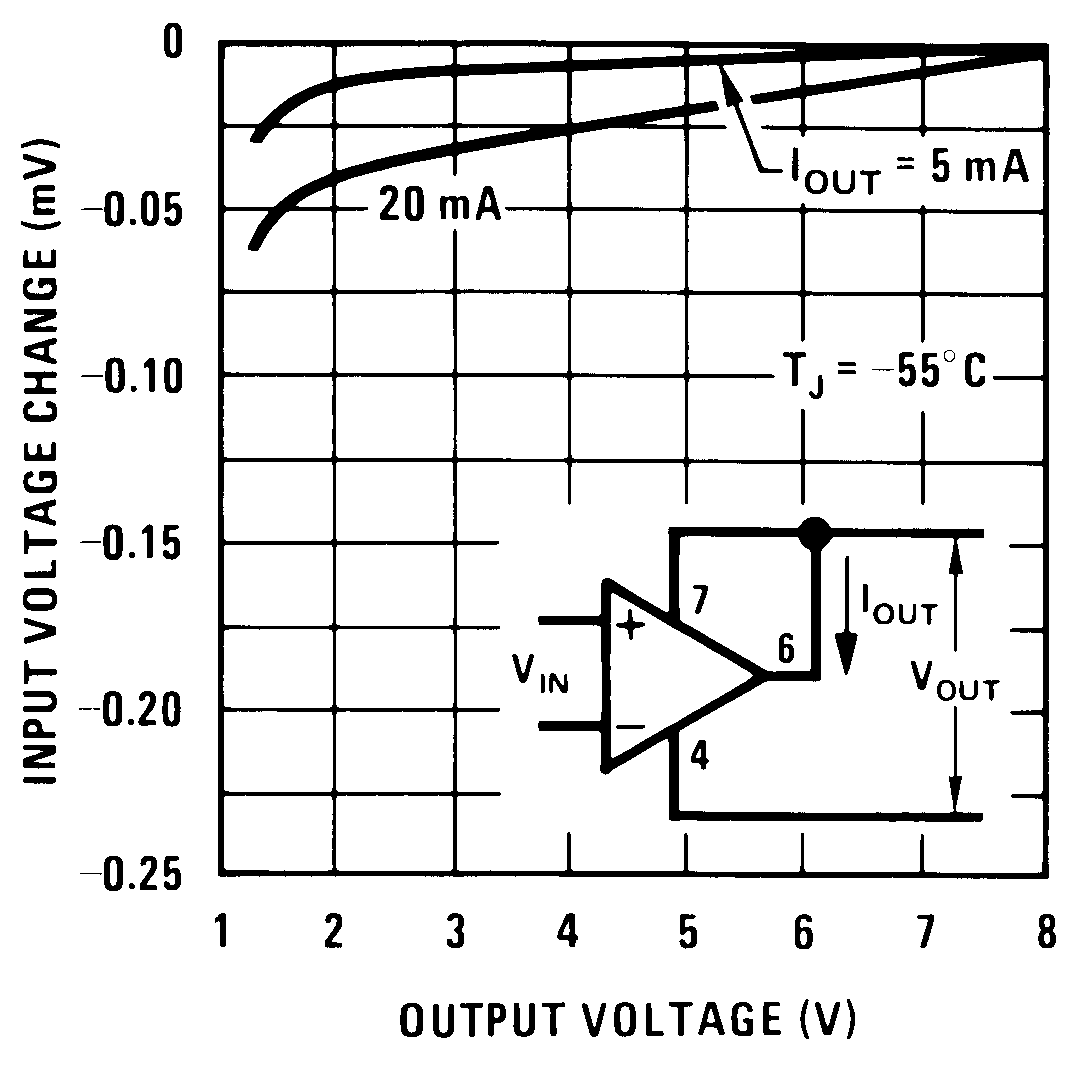Figure 27. Shunt Gain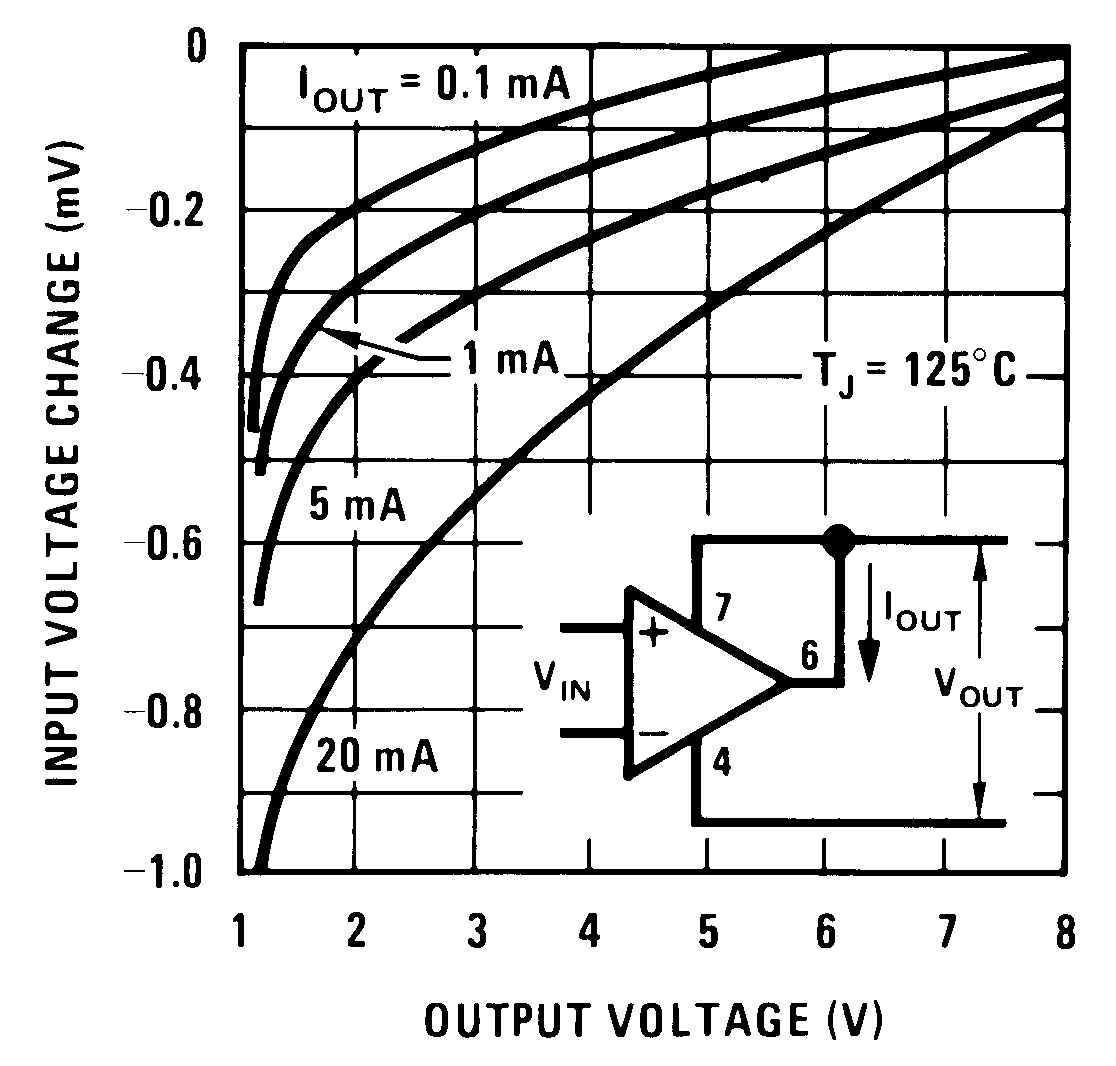Figure 29. Shunt GainFigure 2. Common-Mode Limits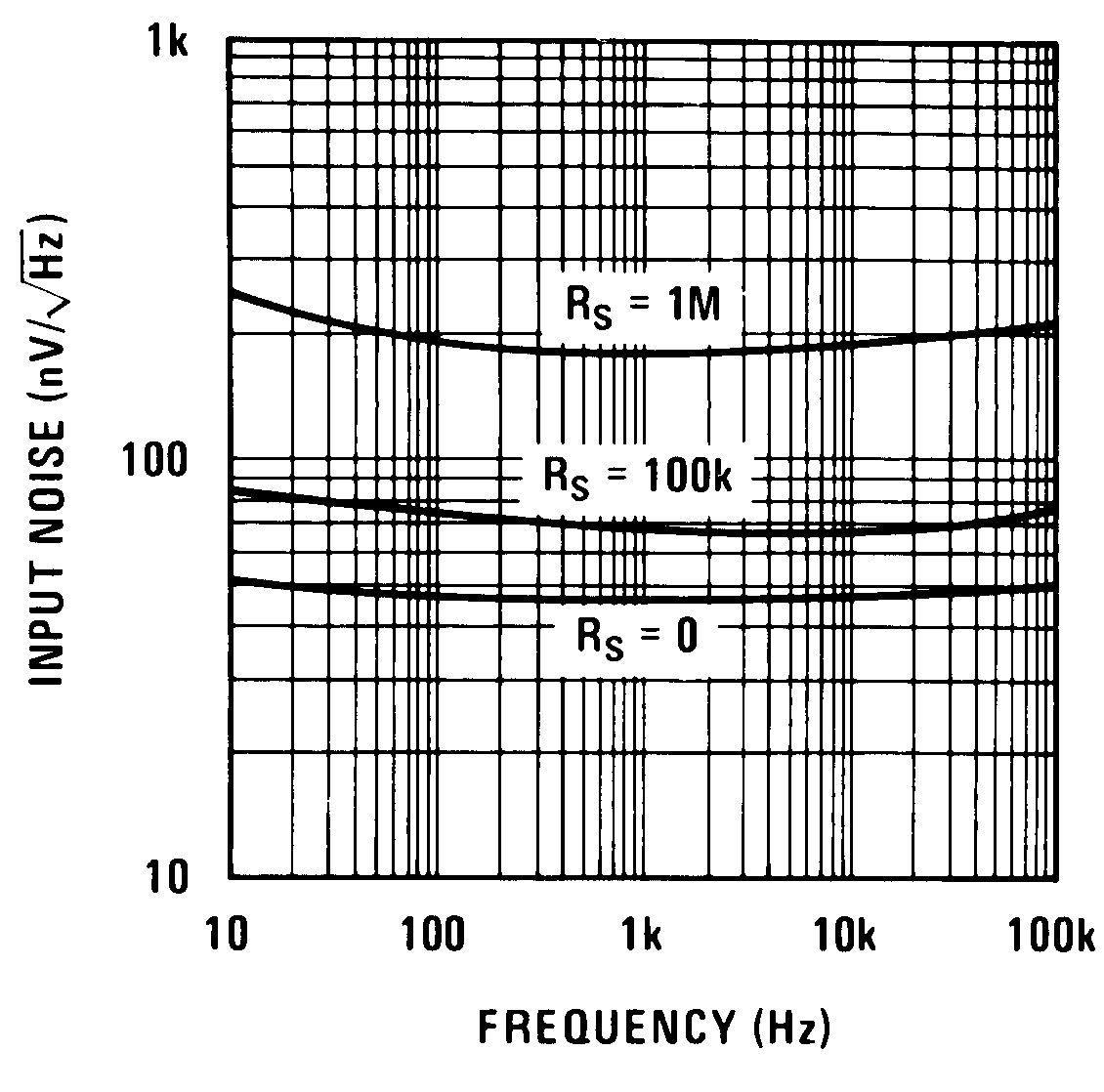Figure 4. Input Noise Voltage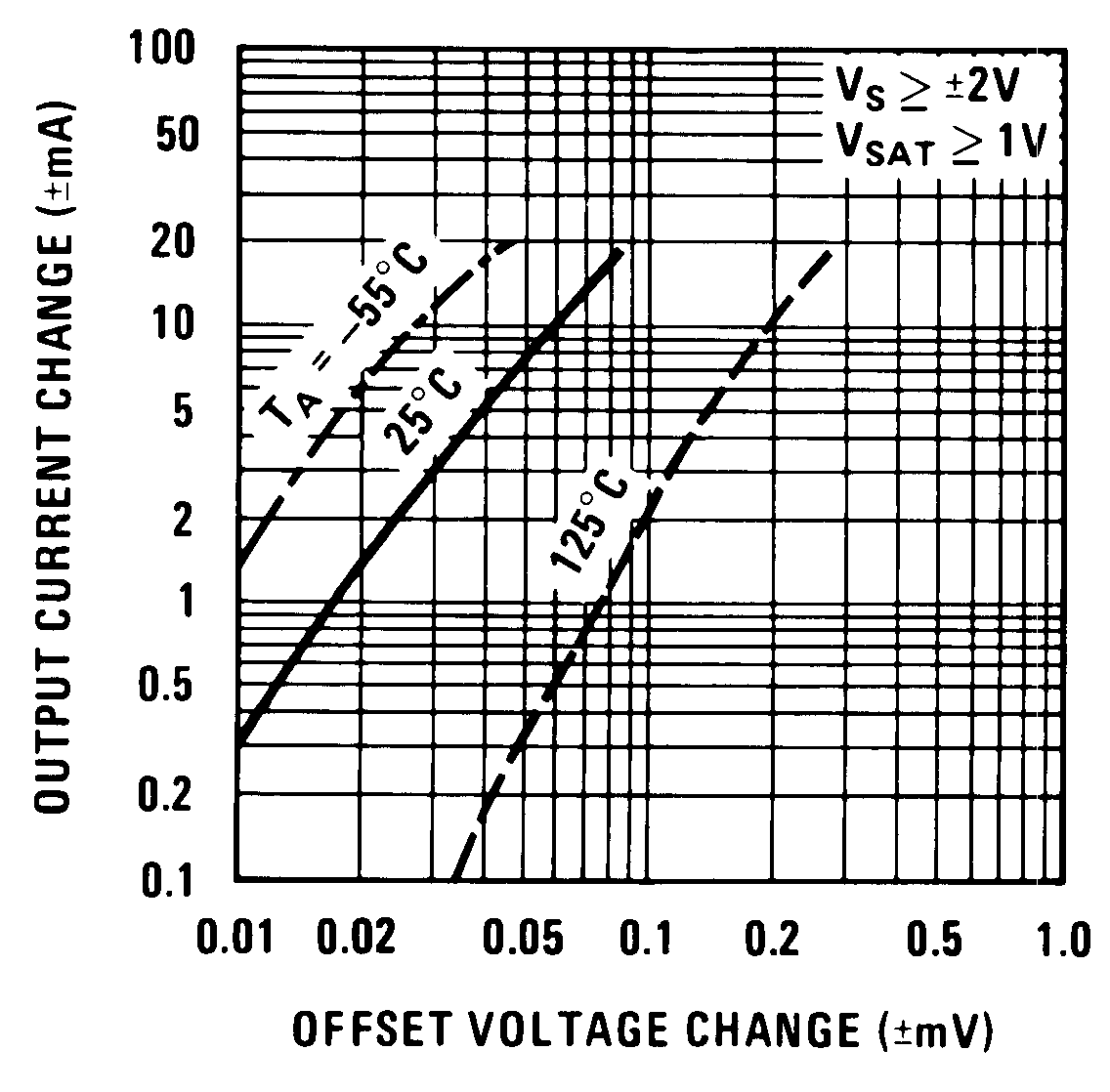Figure 6. Transconductance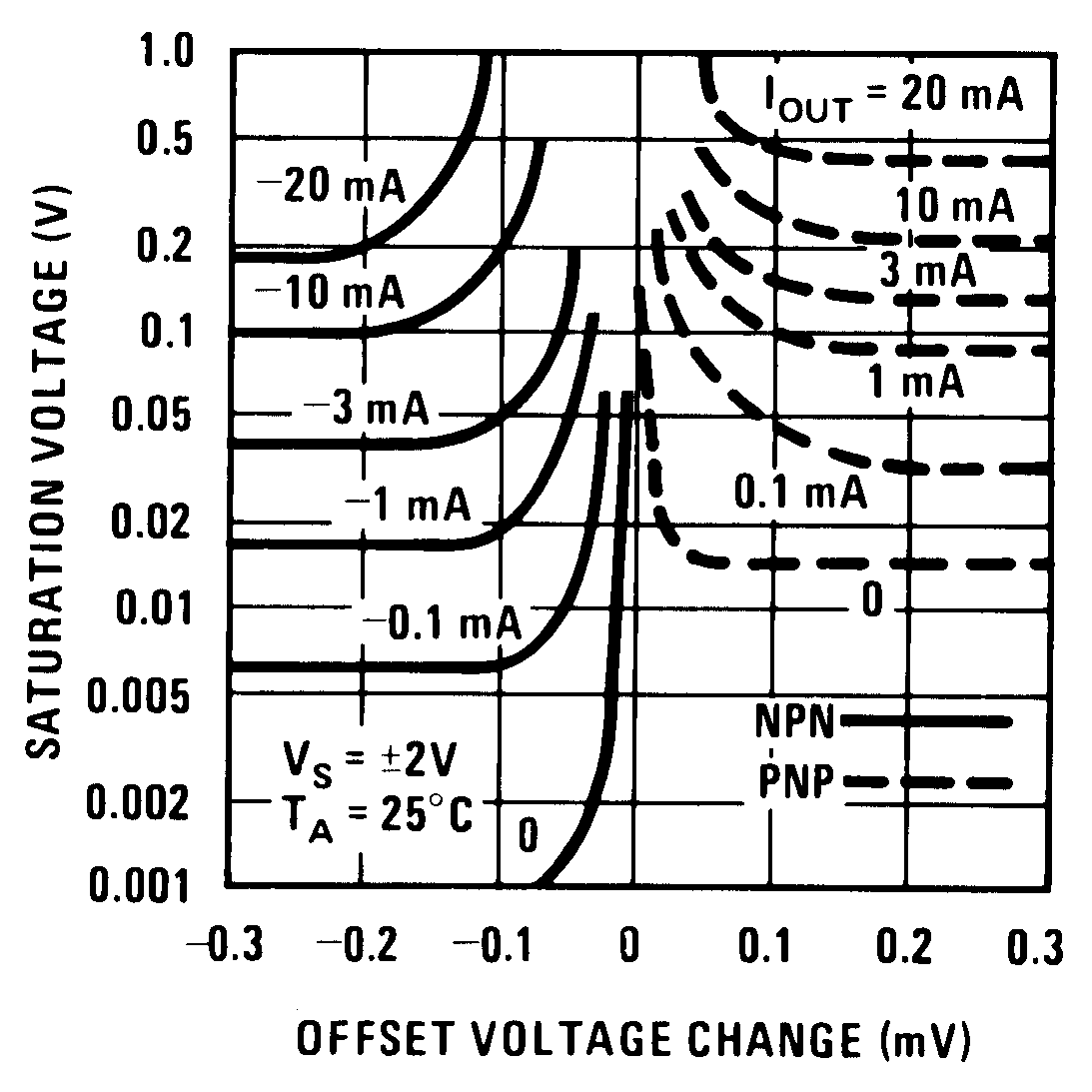Figure 8. Output Saturation Characteristics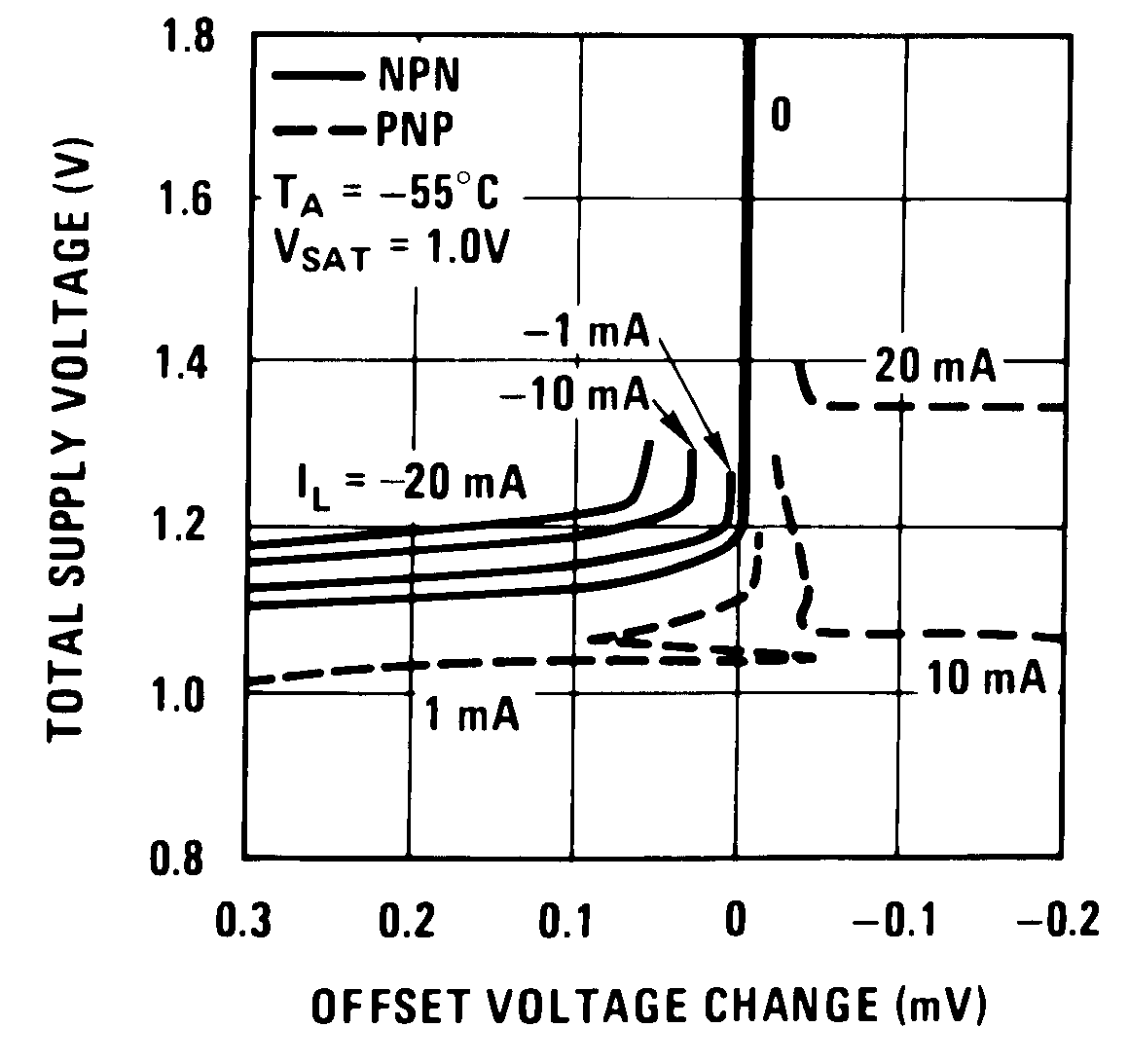Figure 10. Minimum Supply Voltage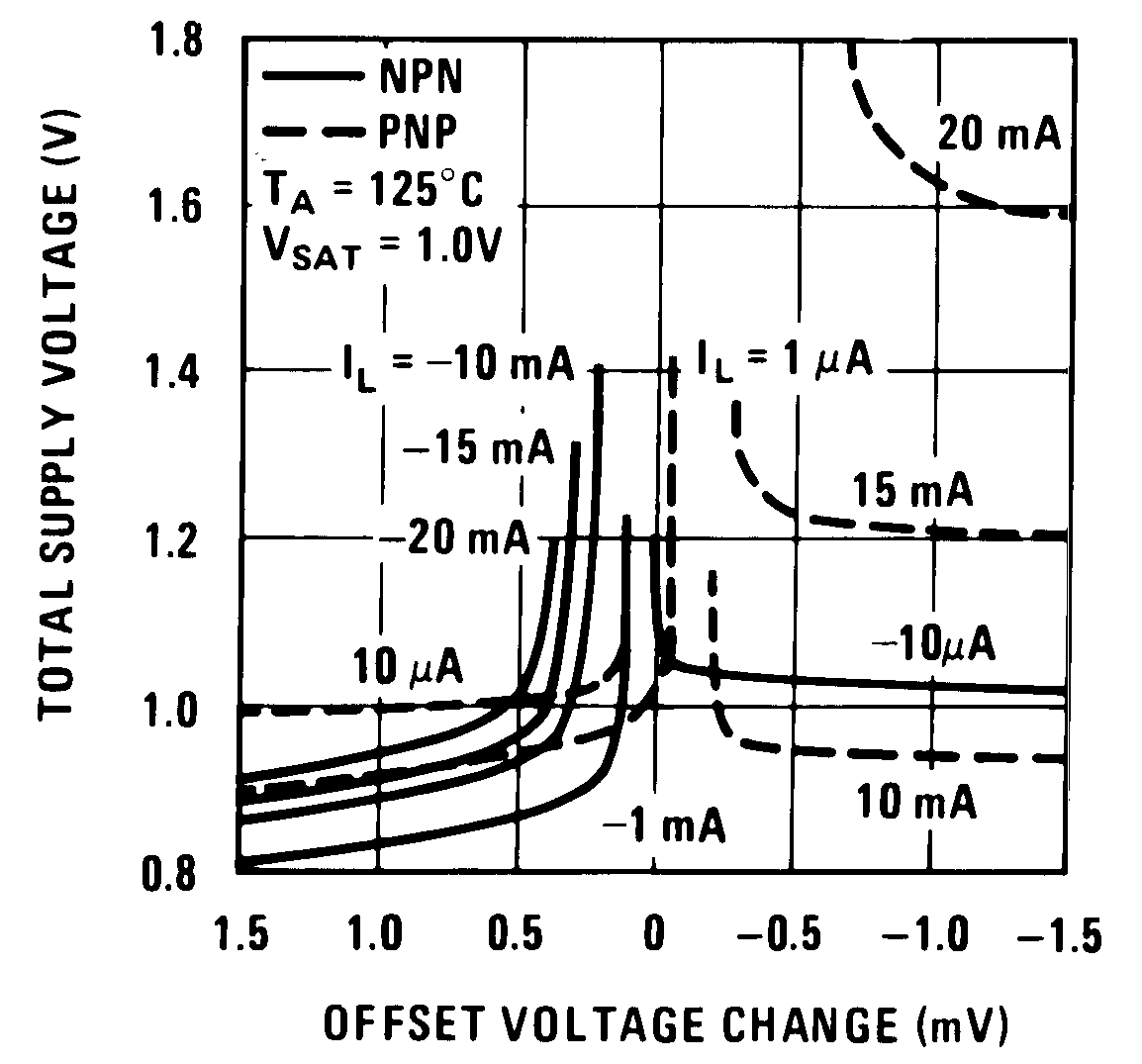Figure 12. Minimum Supply Voltage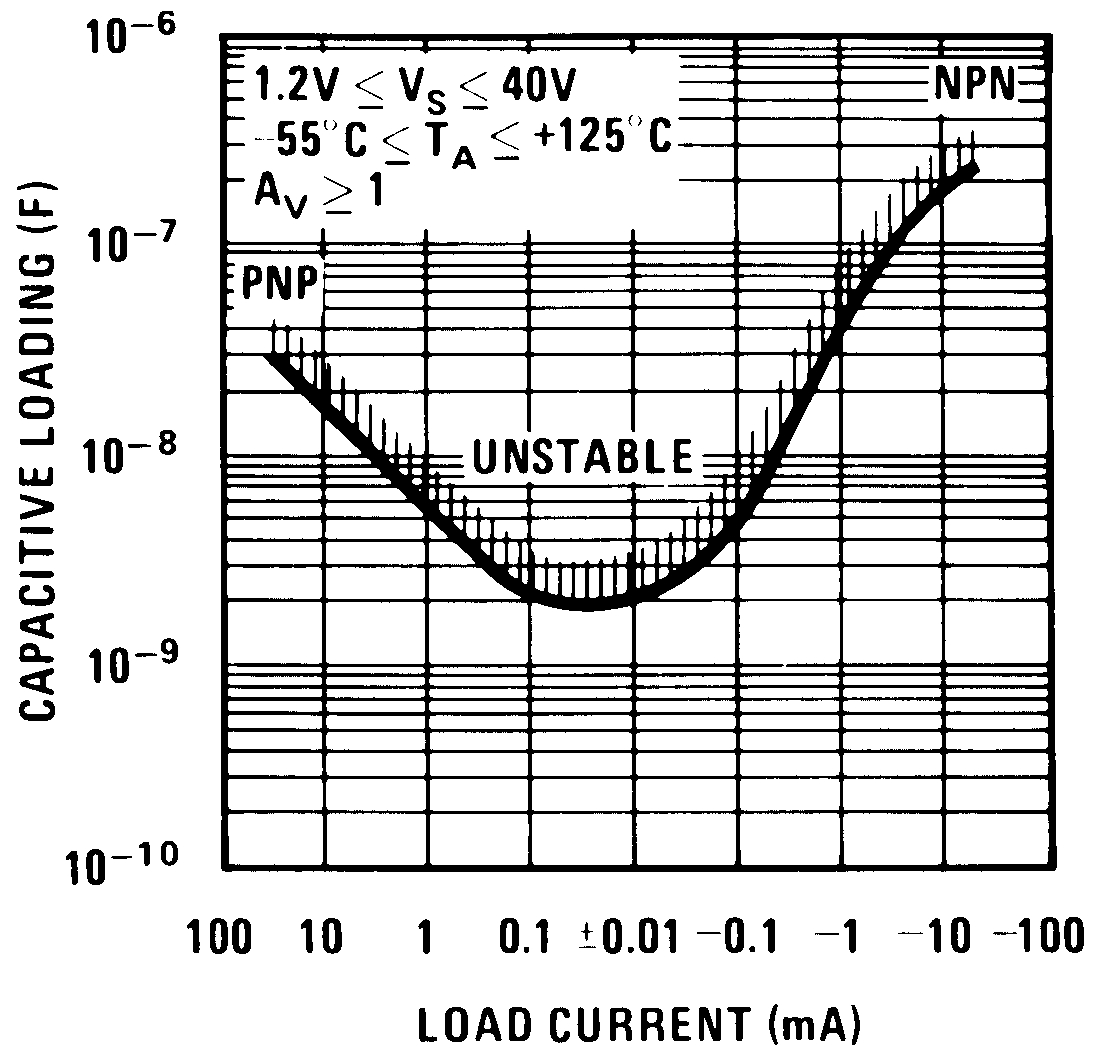Figure 14. Typical Stability Range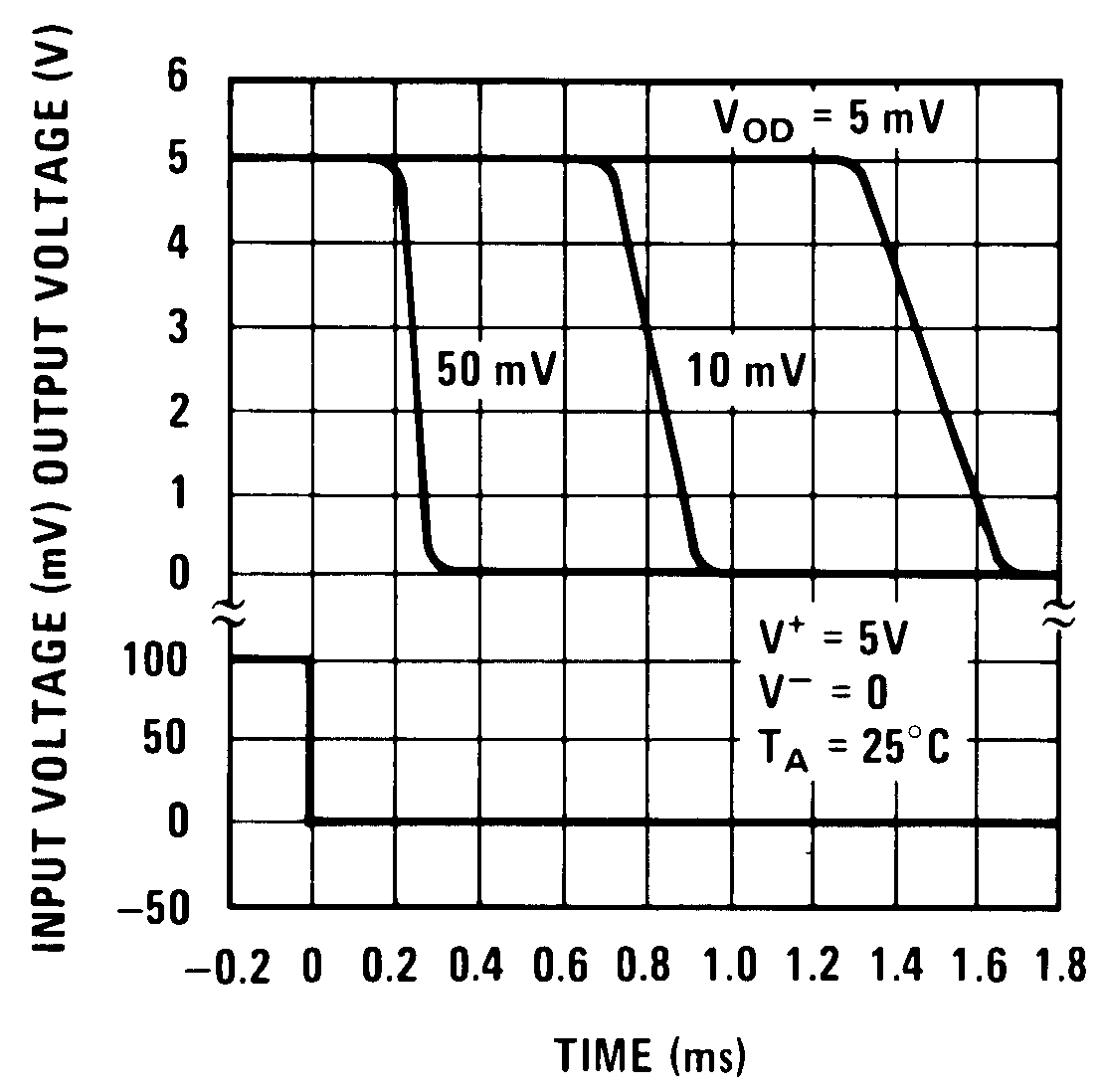Figure 16. Comparator Response Time For Various Input Overdrives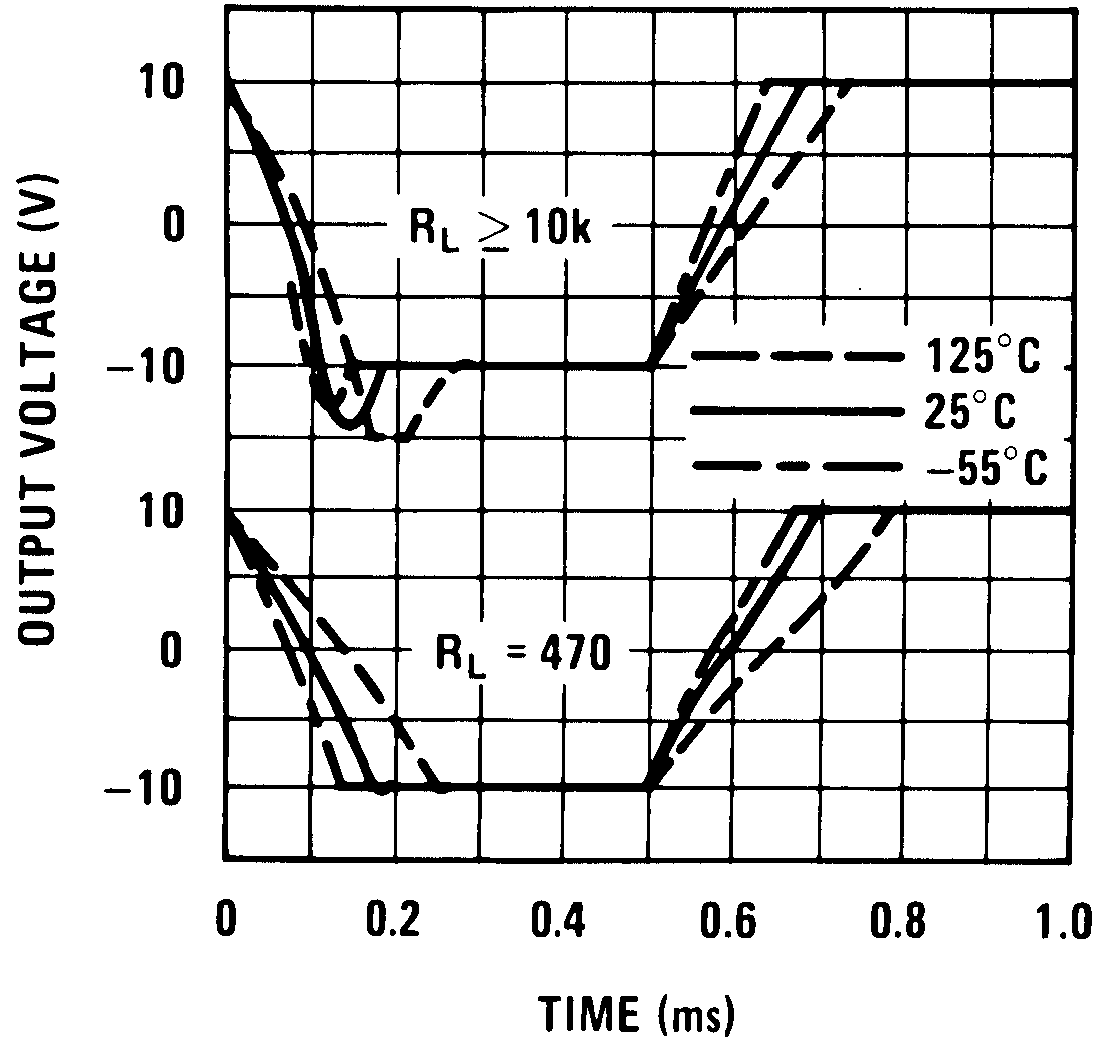Figure 18. Follower Pulse Response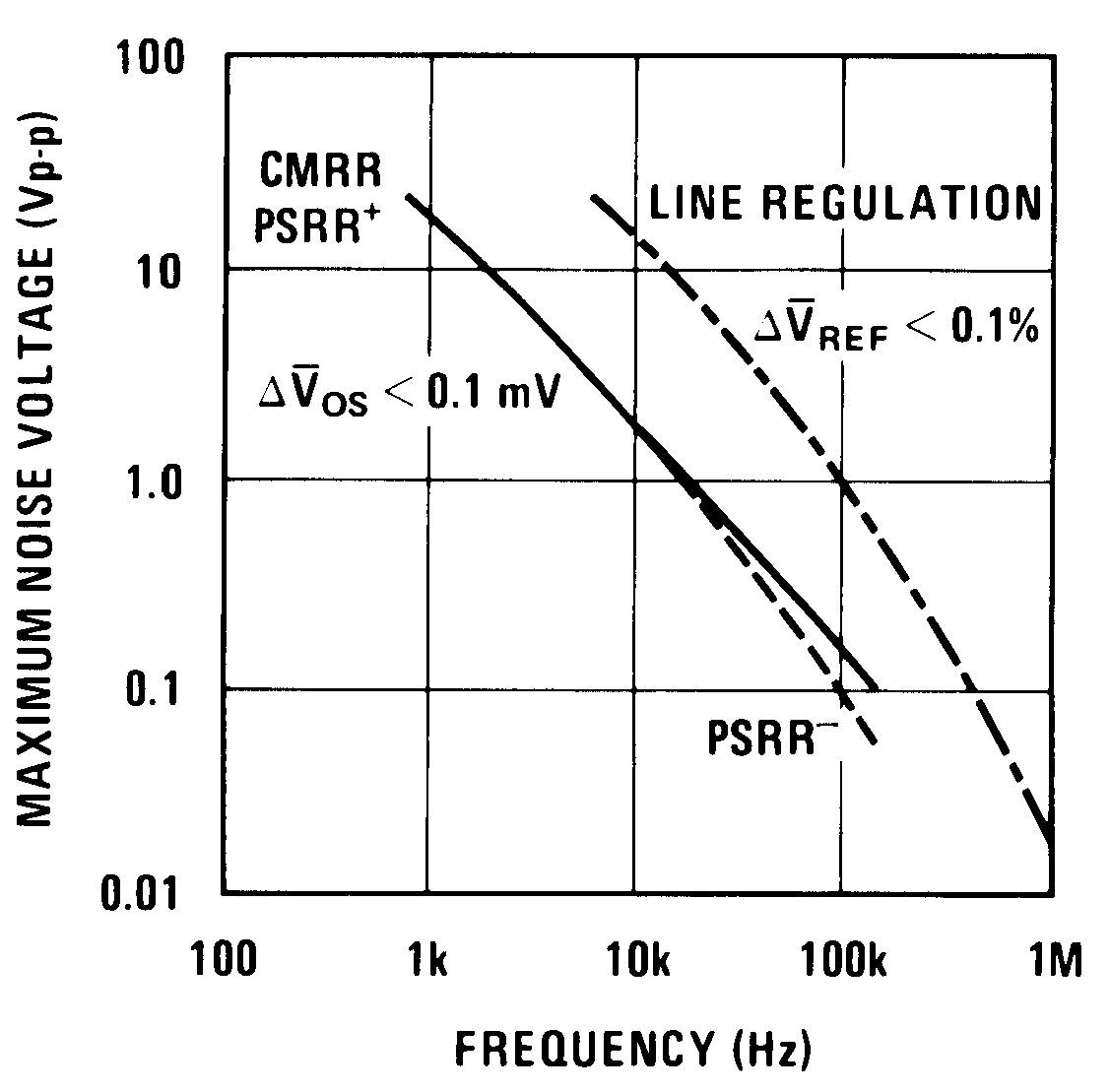Figure 20. Rejection Slew LimitingFigure 24. Shunt GainFigure 26. Shunt GainFigure 28. Shunt Gain

#### 6.8.2 Typical Characteristics (Reference)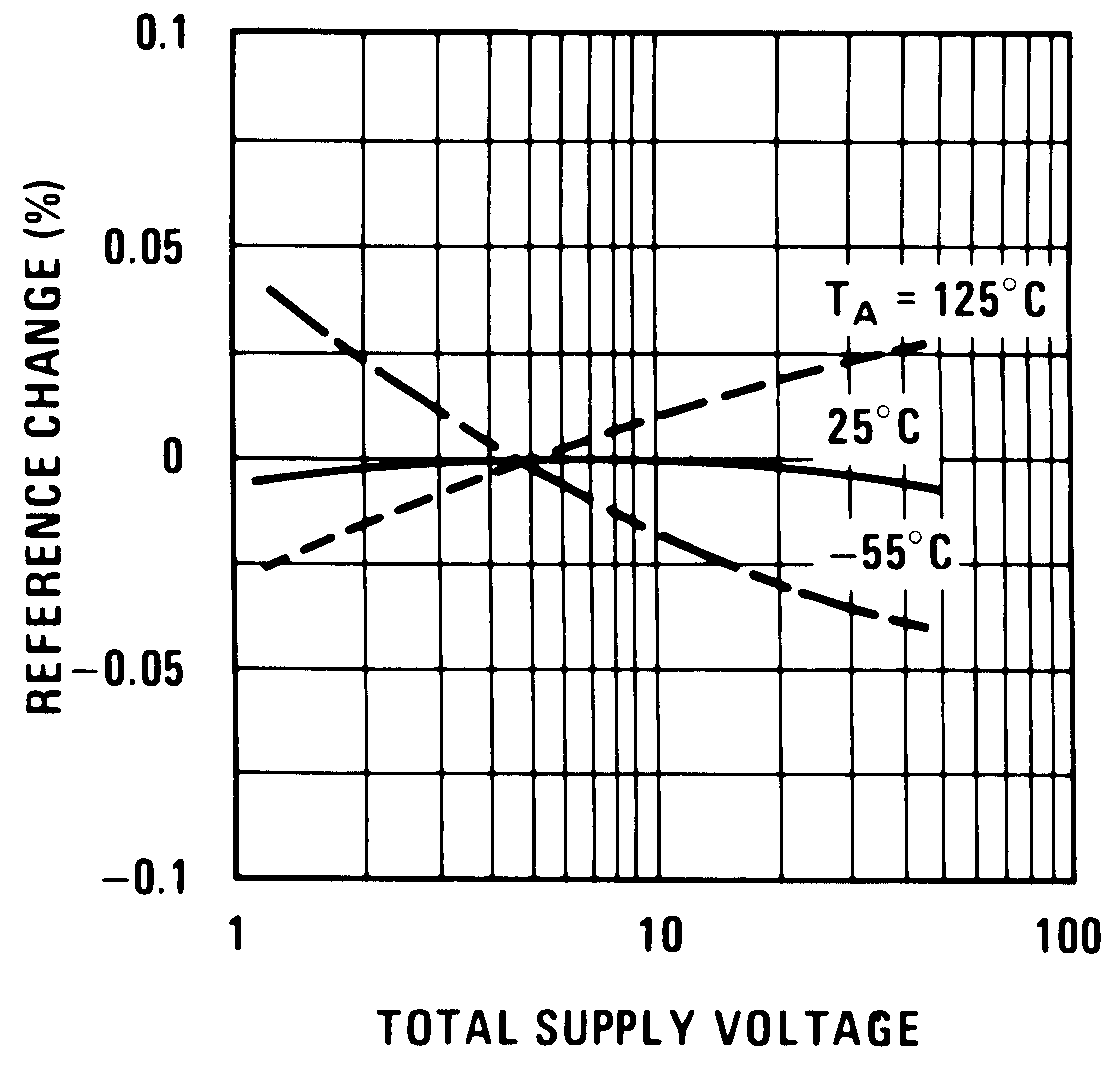Figure 30. Line Regulation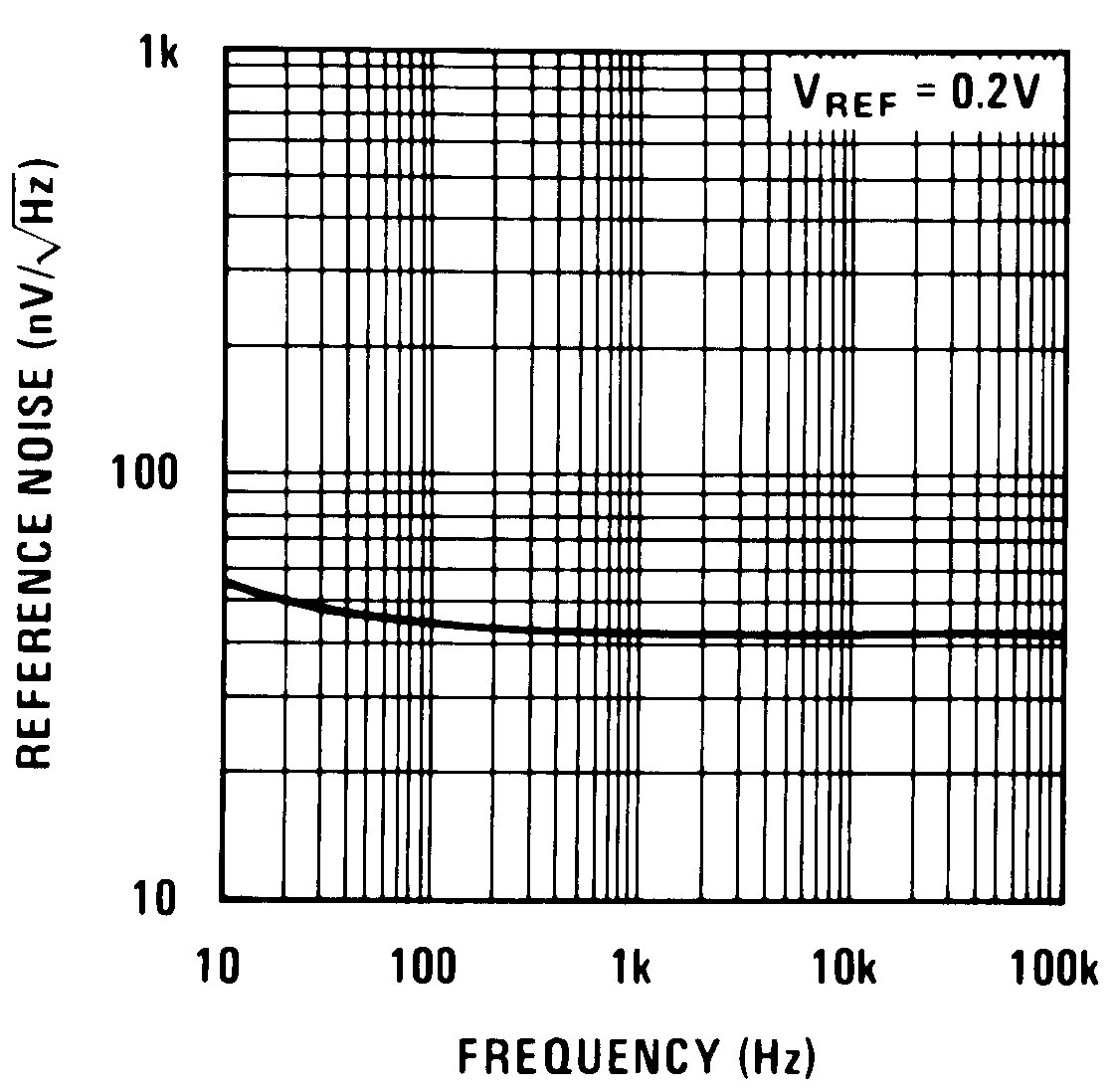Figure 32. Reference Noise Voltage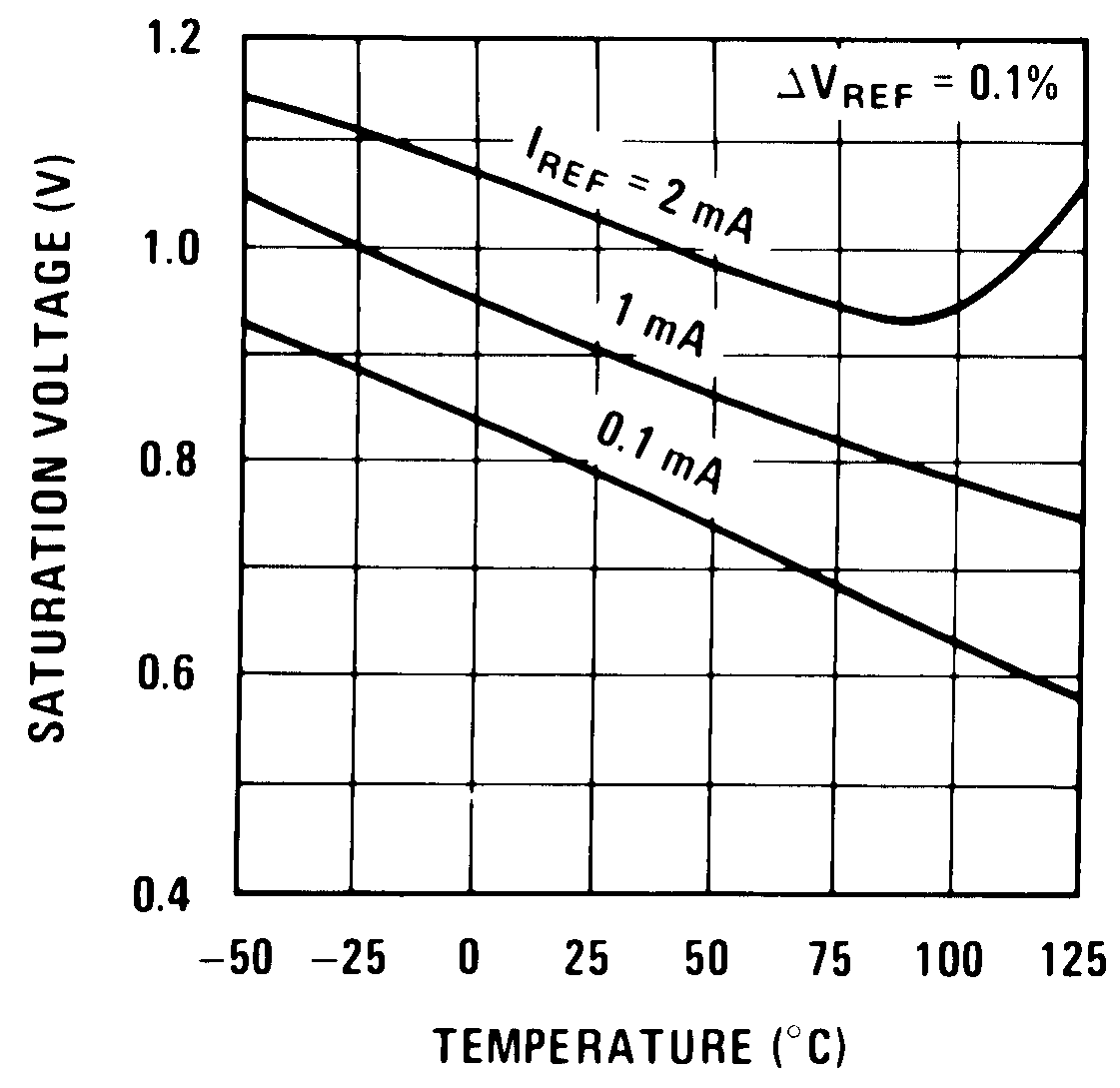Figure 34. Output Saturation